# ML Aggarwal Solutions for Class 9 Maths Chapter 9 - Logarithms

ML Aggarwal Solutions for Class 9 Maths Chapter 9 – Logarithms are provided here to help students prepare for their exams and score well. This chapter mainly deals with problems based on logarithms. Solutions are prepared by our experienced faculty team at BYJU’S, which are created for the purpose of clarifying student’s doubts, as per their convenience. These solutions also provide guidance to students for solving problems confidently, which in turn, helps in improving their problem-solving skills. This is very important from the examination point of view. For students wishing to learn the right steps of solving such problems, the ML Aggarwal Solutions is made available in the pdf format. Students can easily download the pdf from the links given below.

Chapter 9 – Logarithms contains two exercises and the ML Aggarwal Class 9 Solutions present in this page provide solutions to questions related to each exercise present in this chapter.

## Download the Pdf of ML Aggarwal Solutions for Class 9 Maths Chapter 9 – Logarithms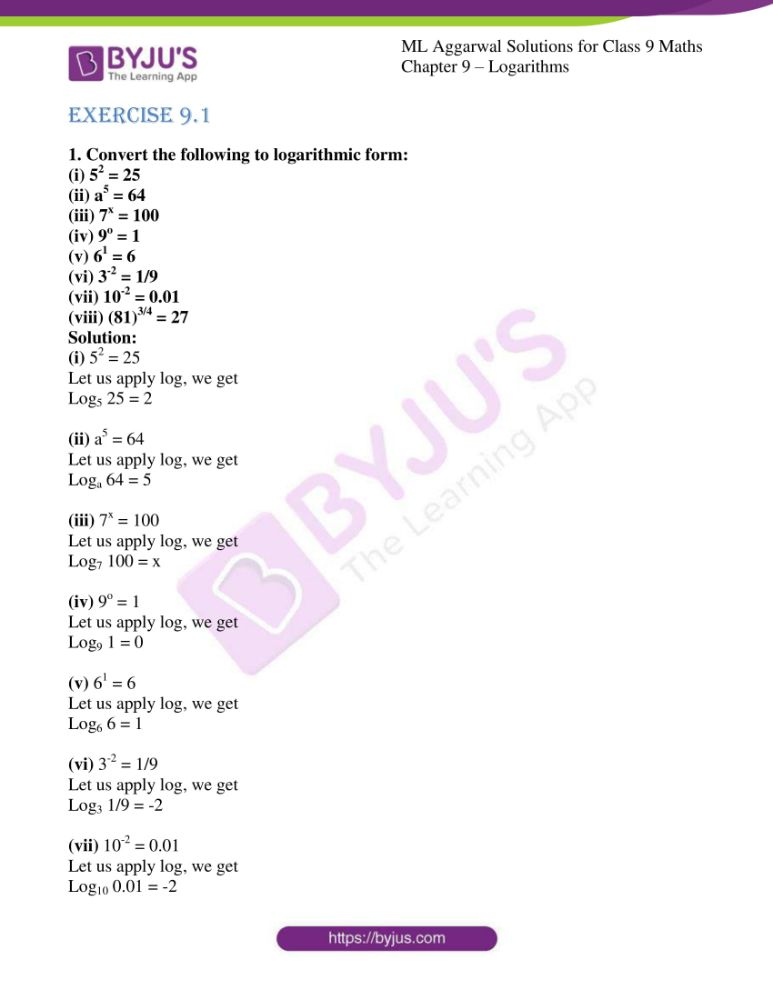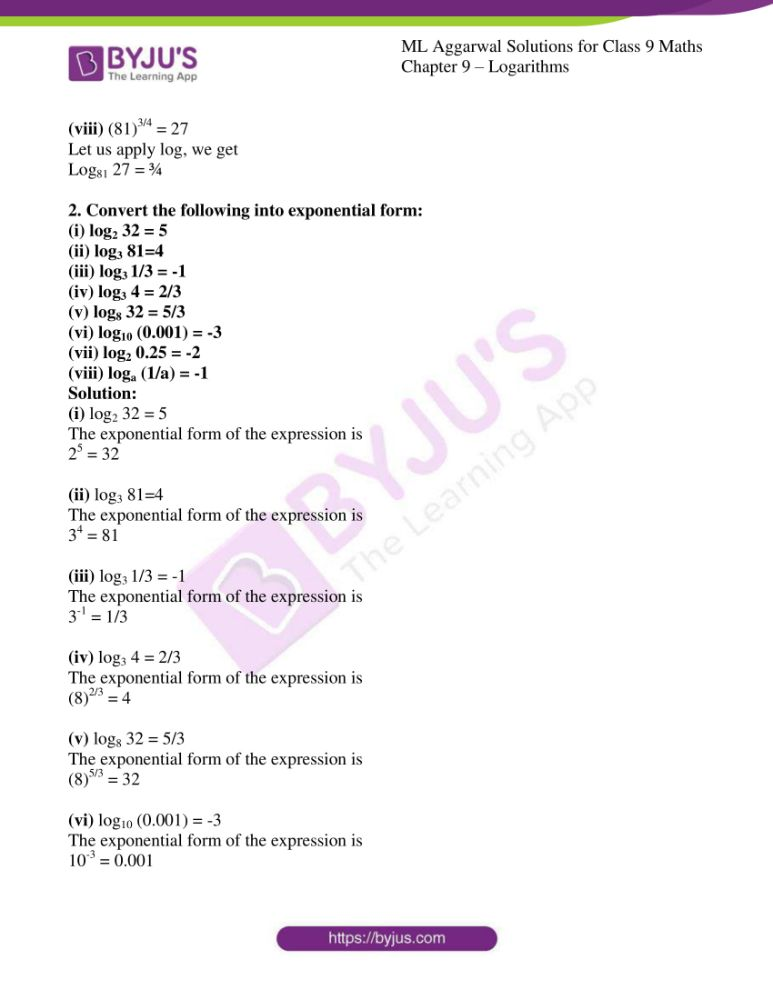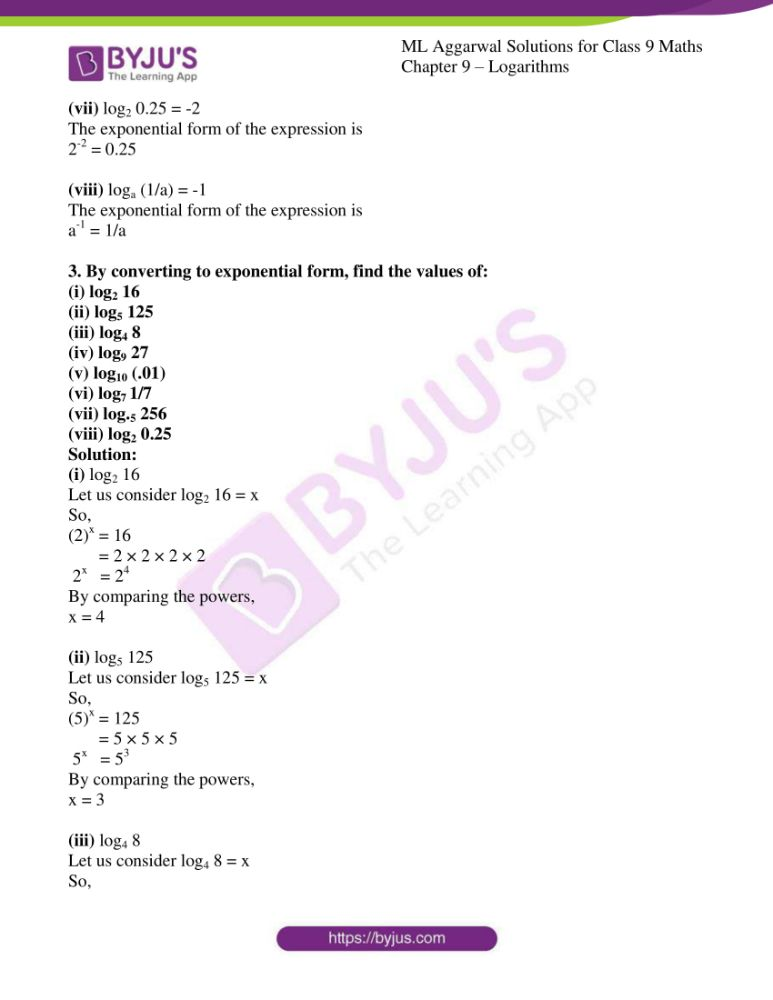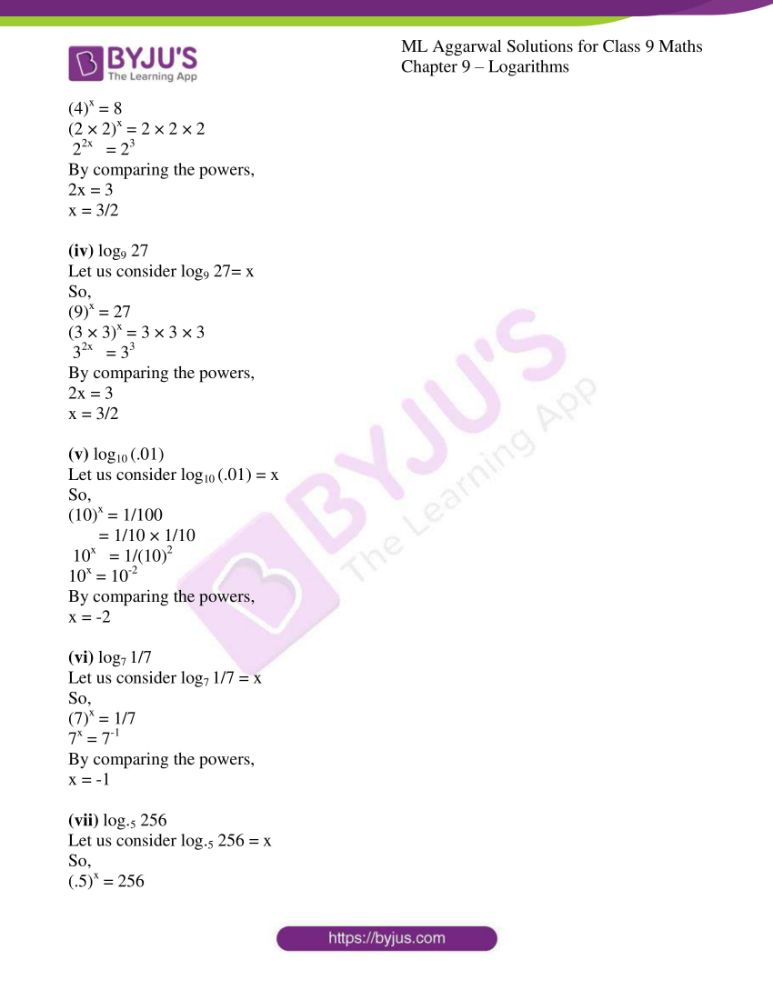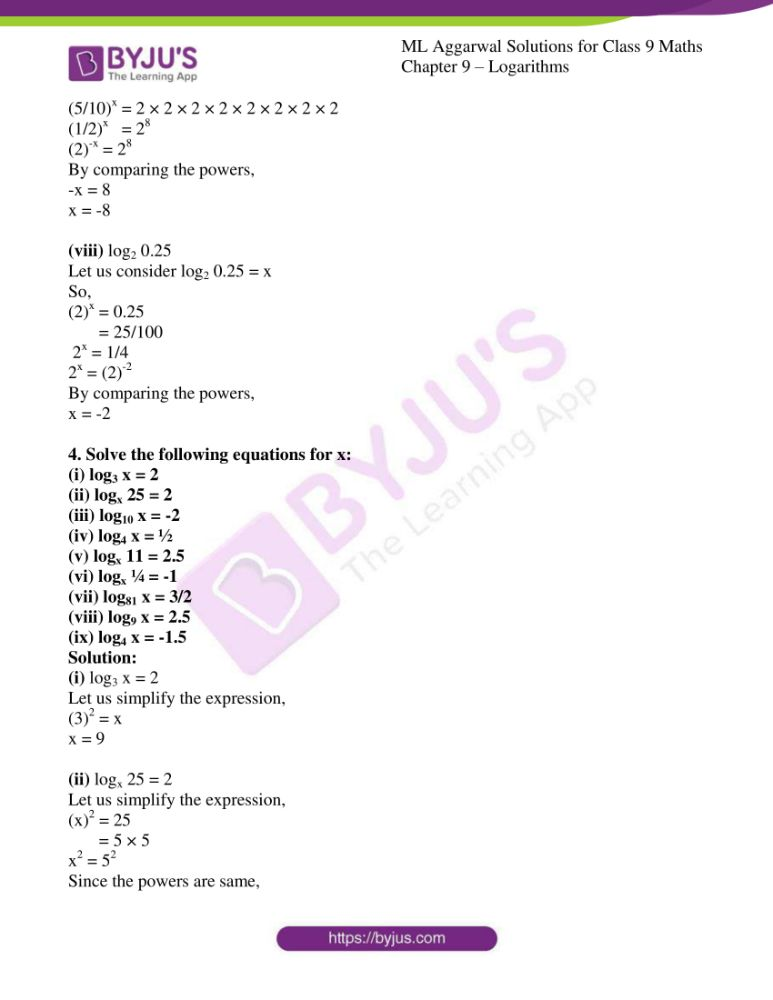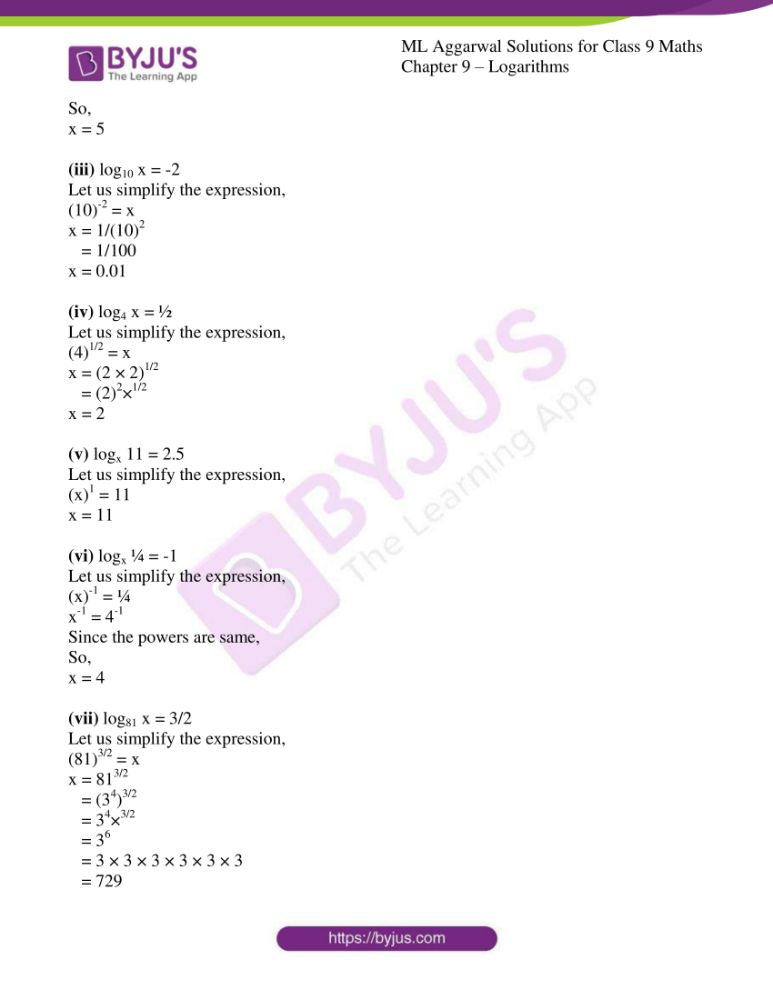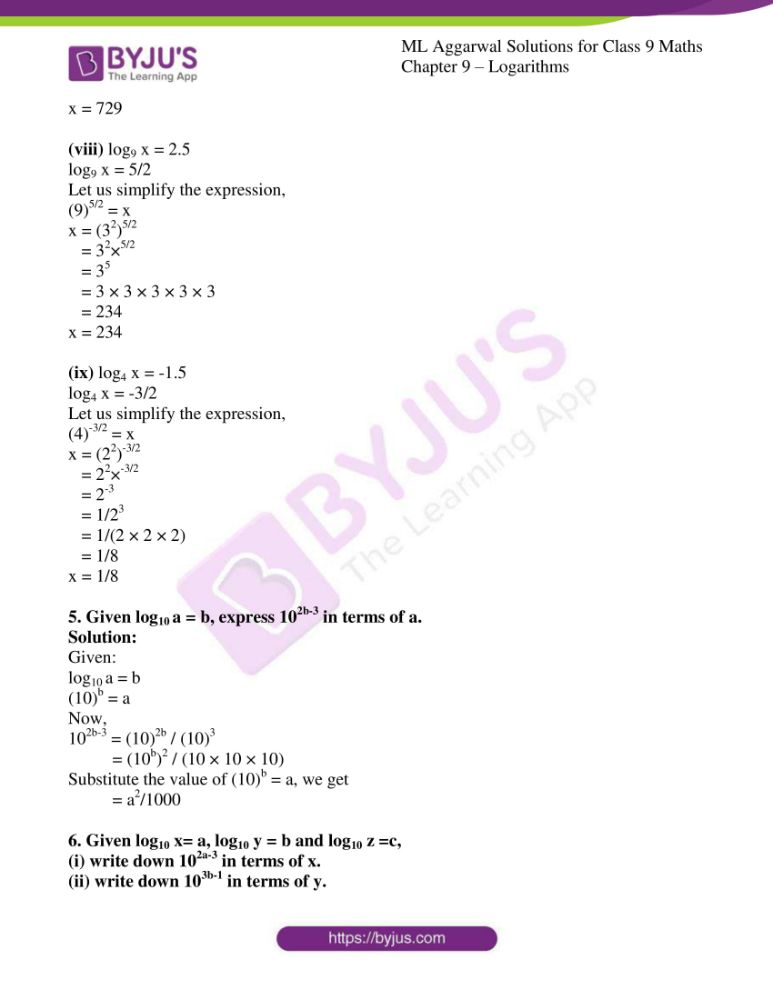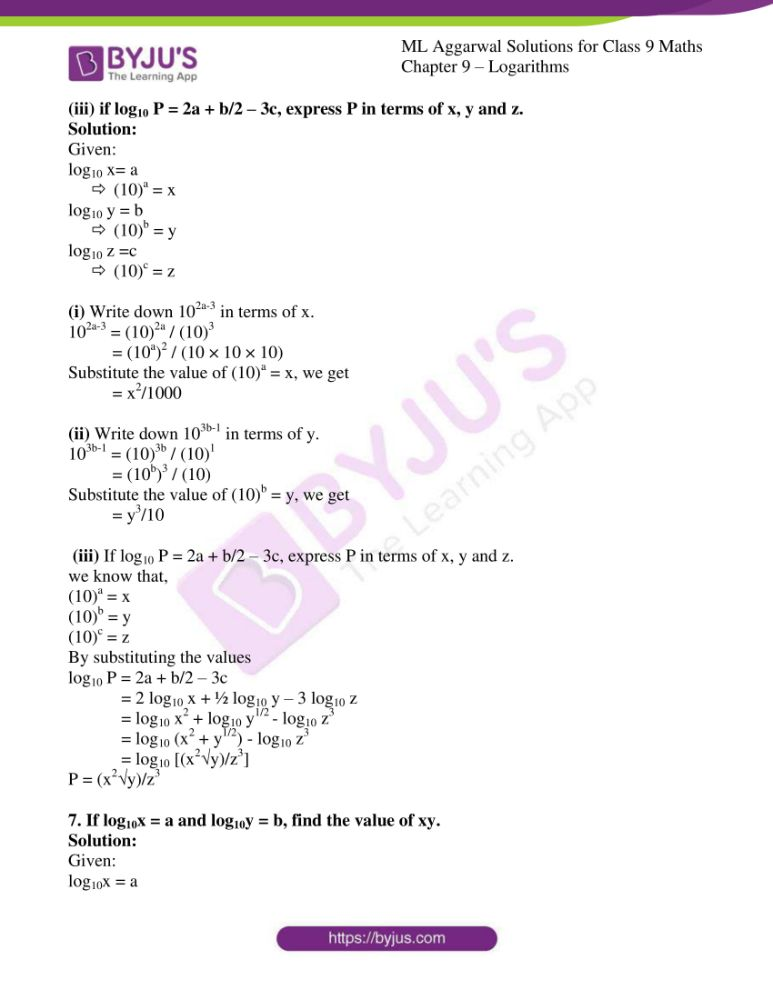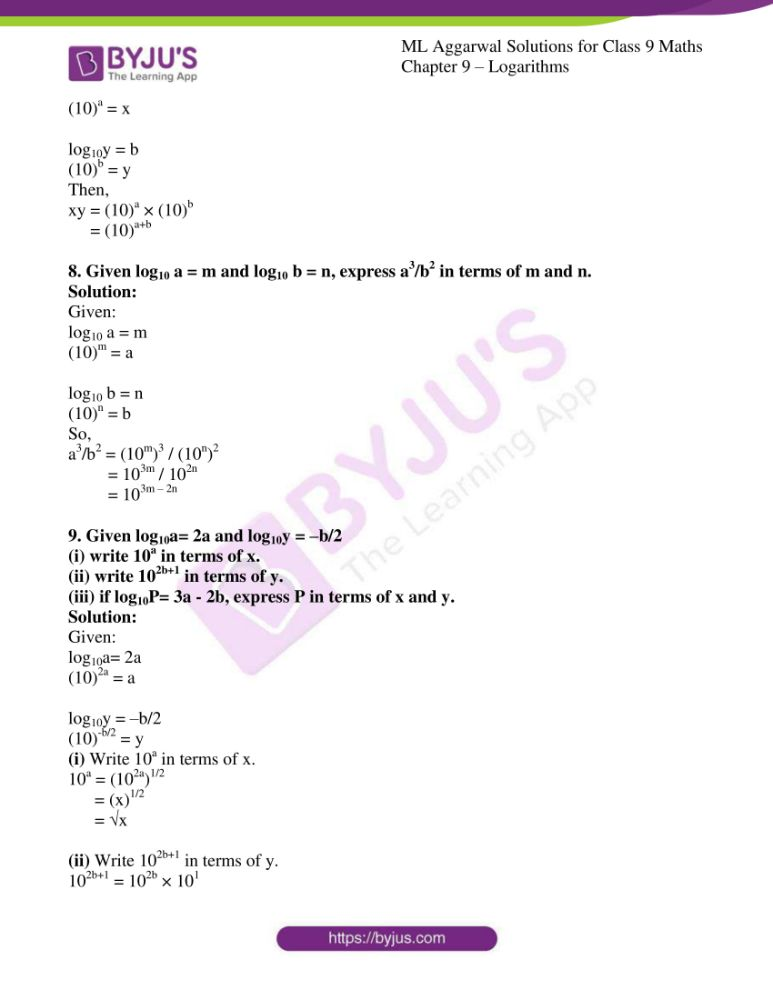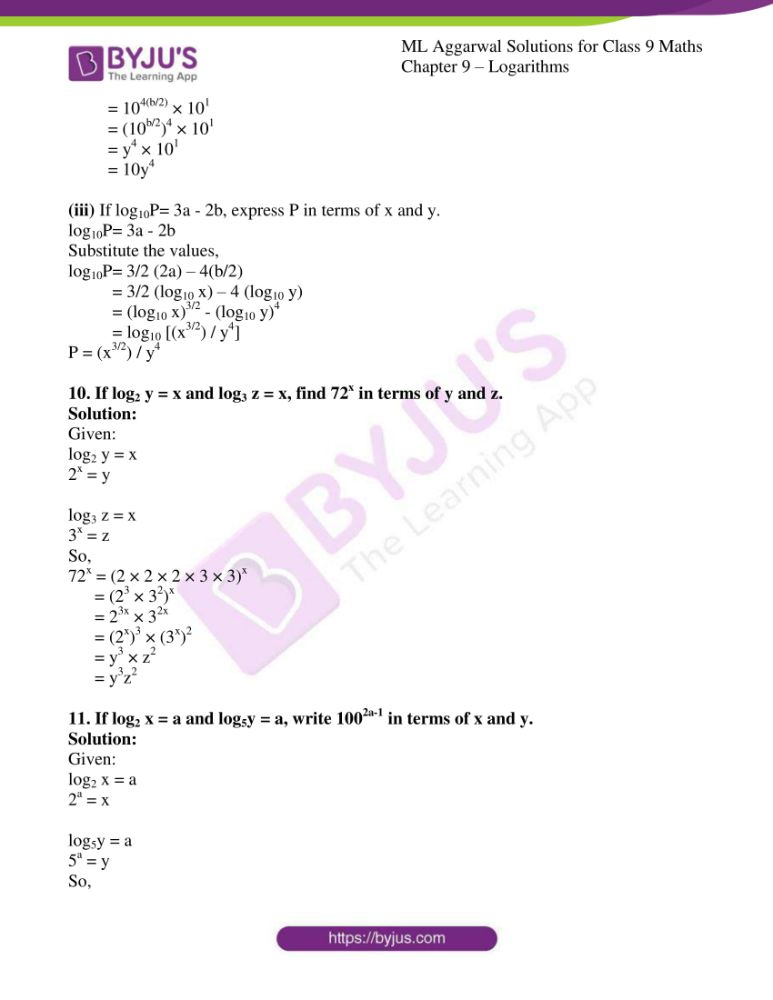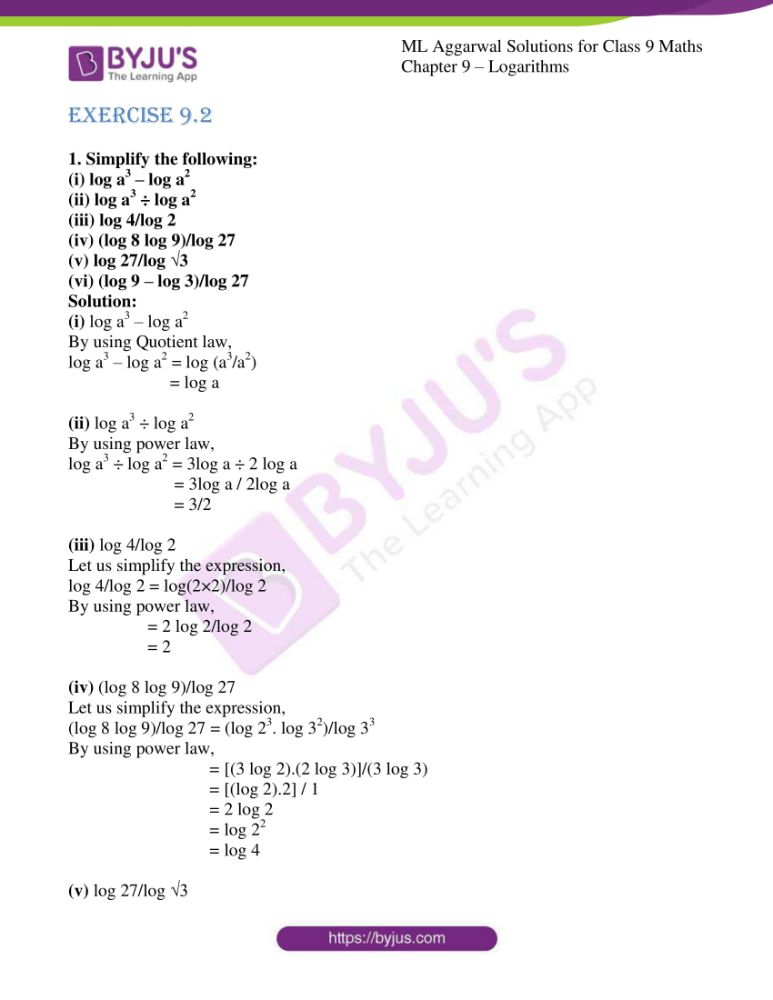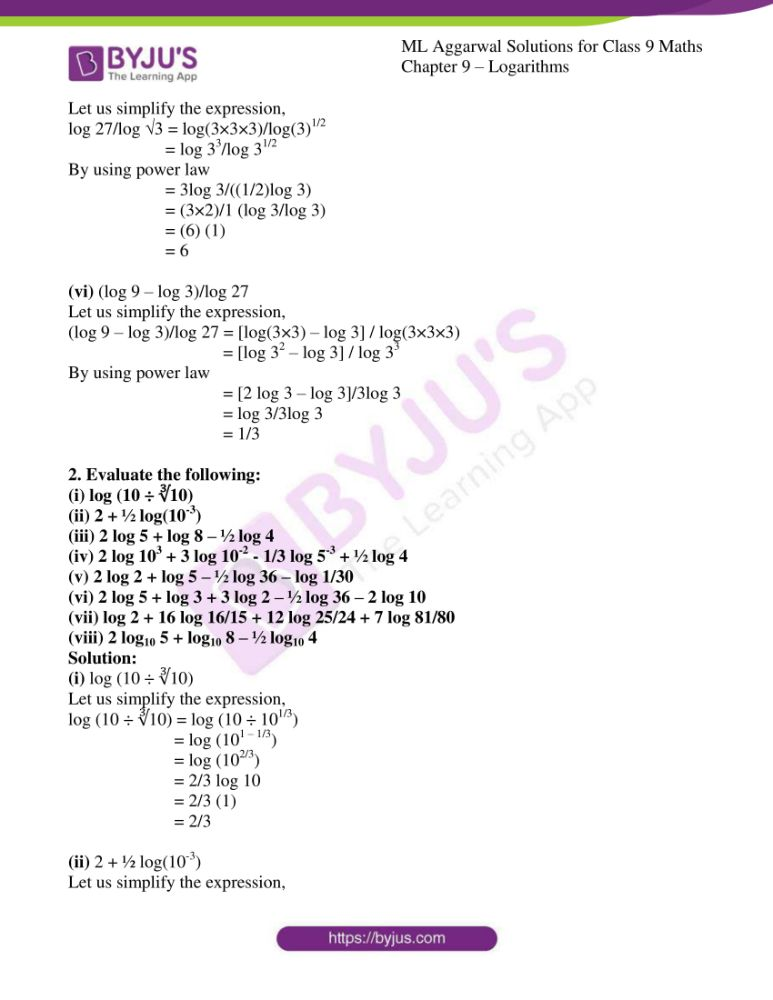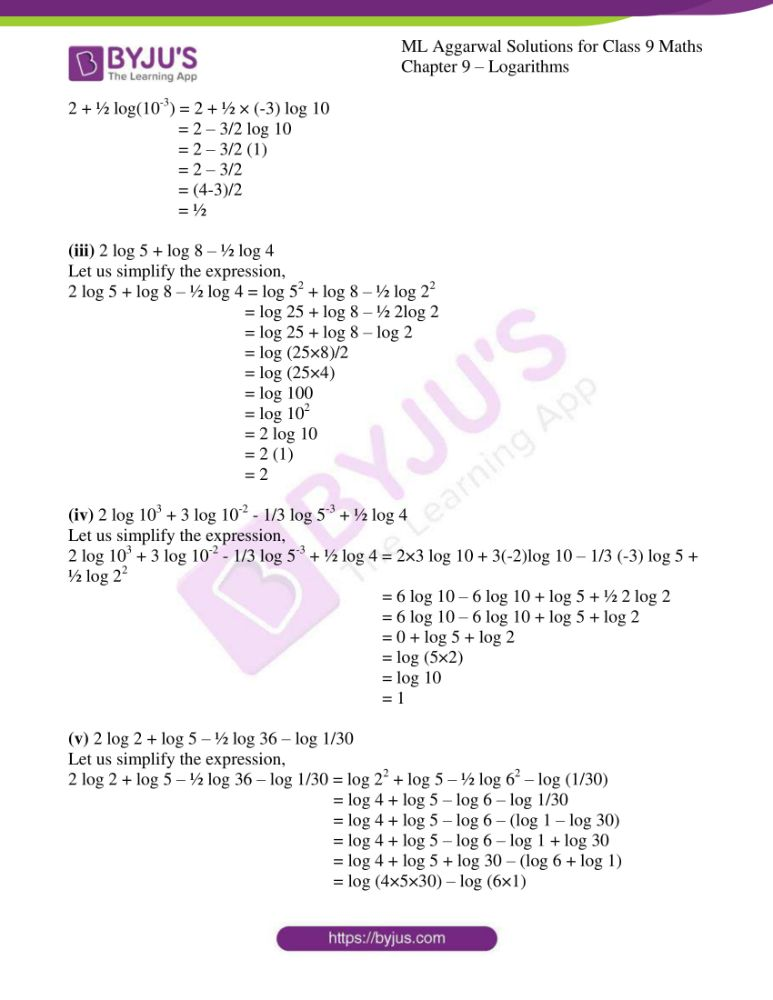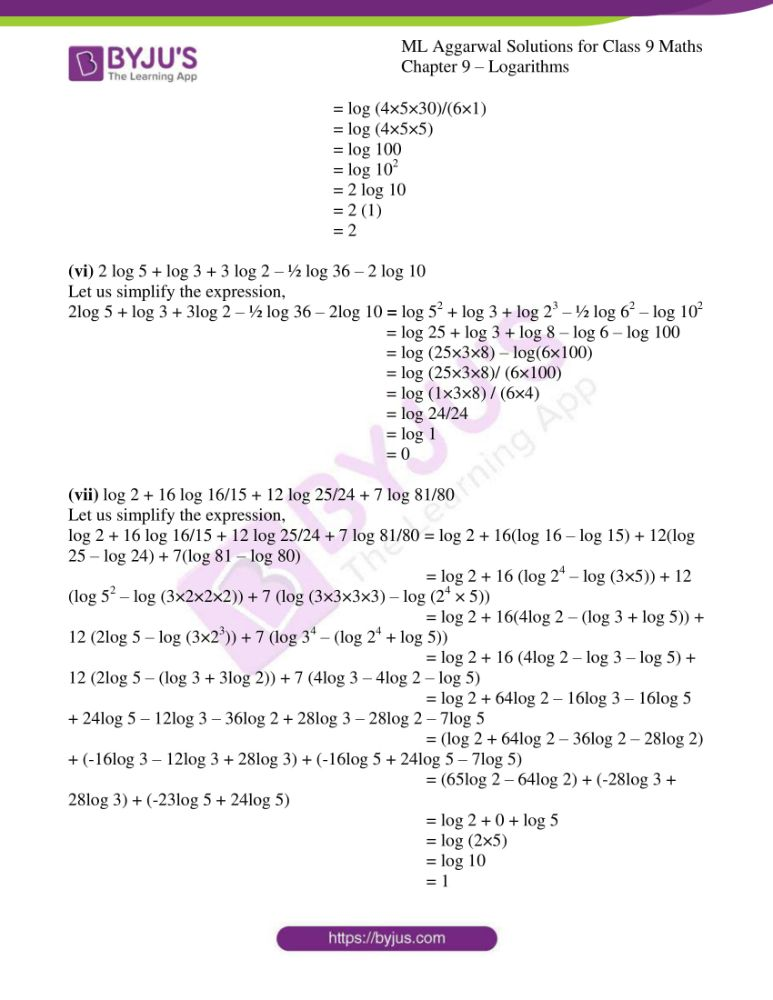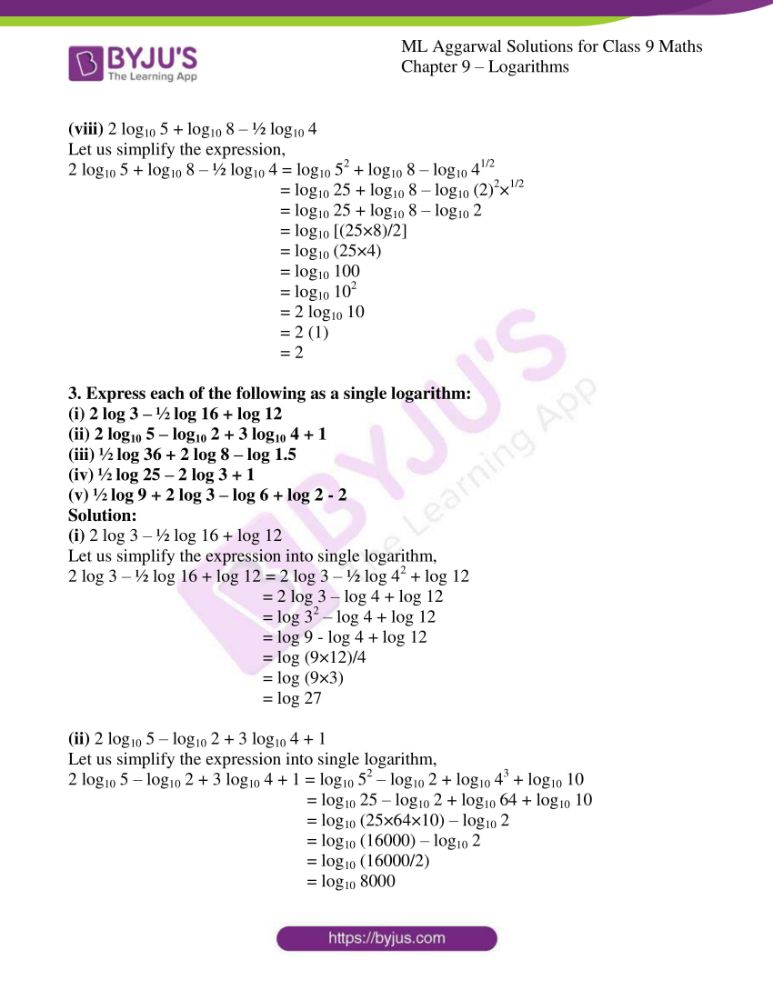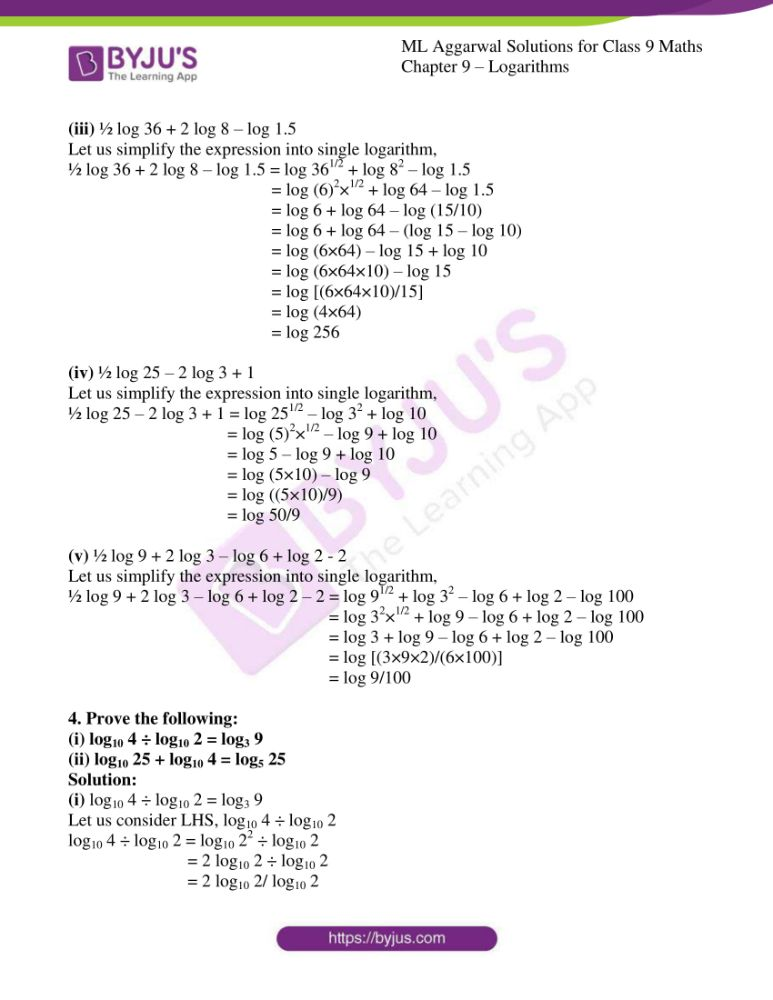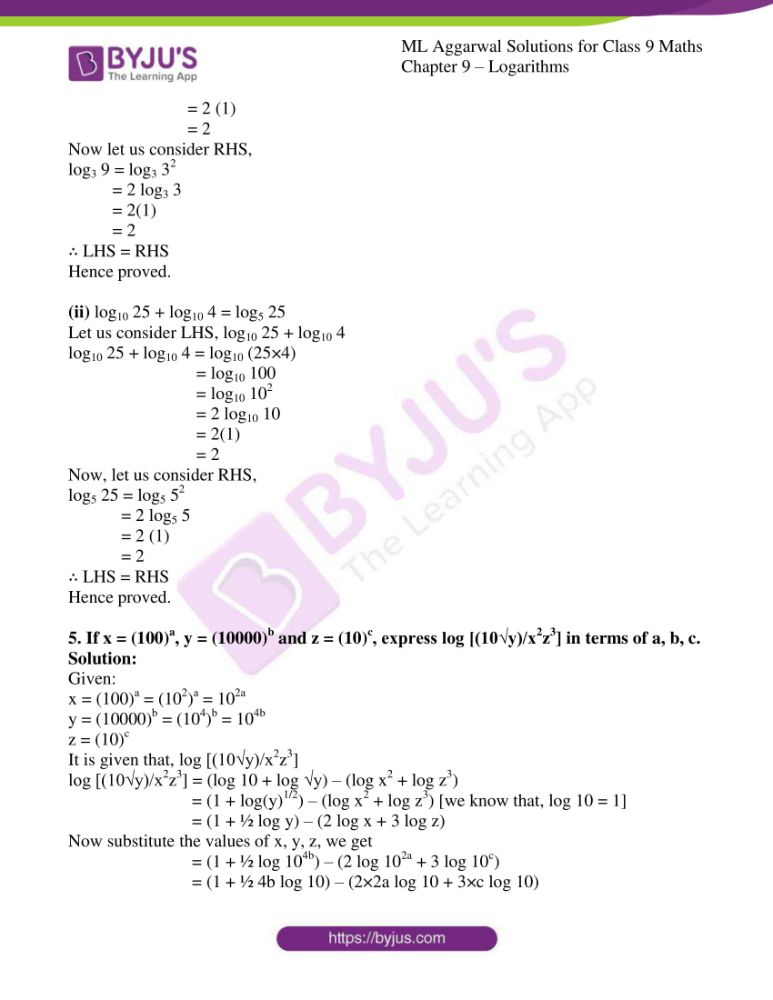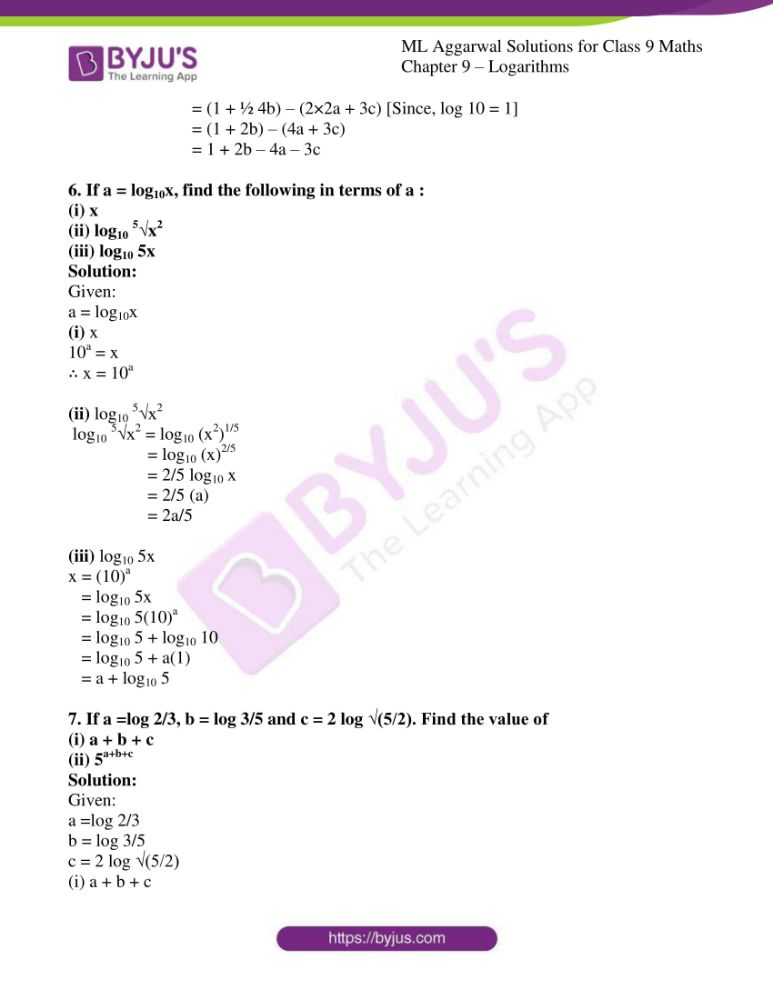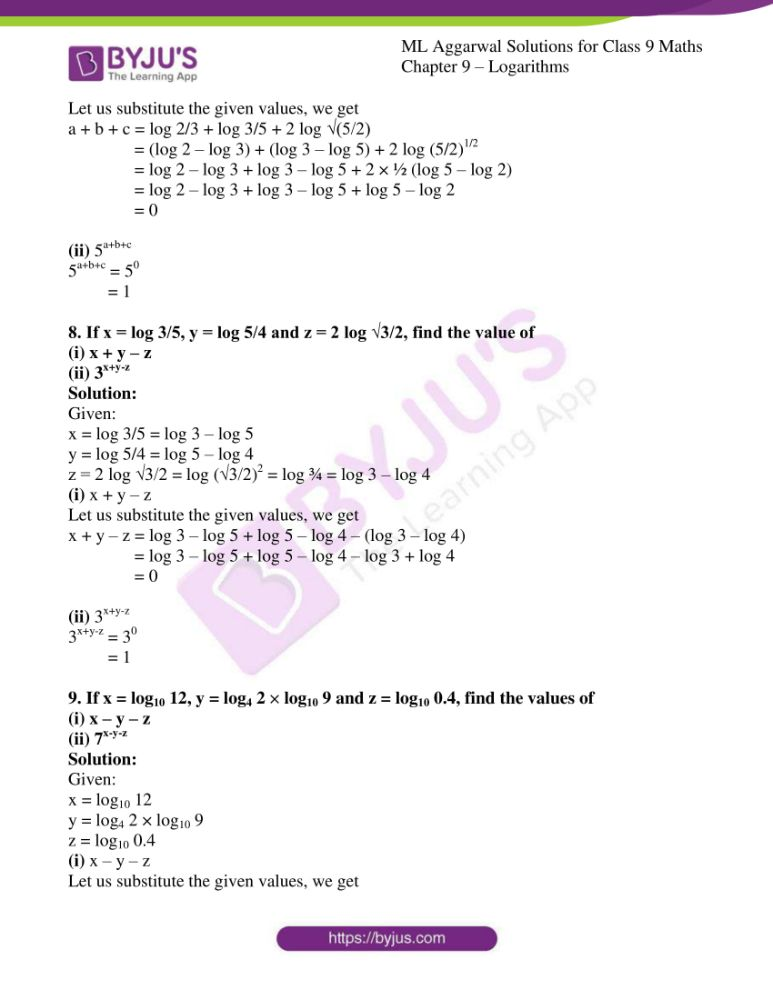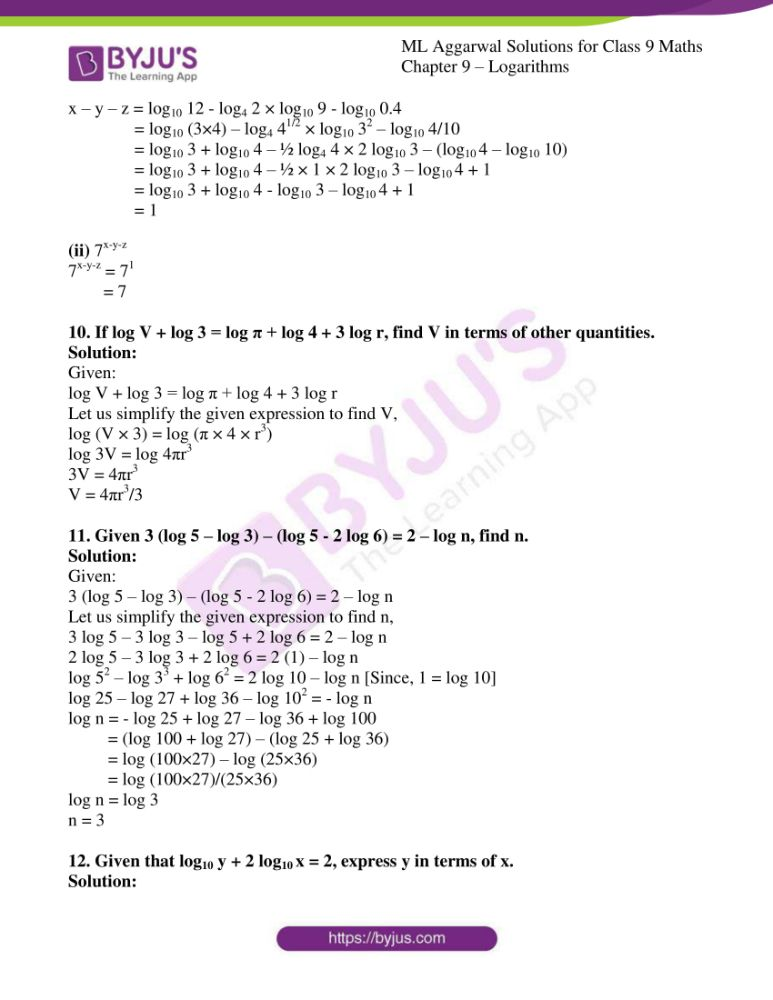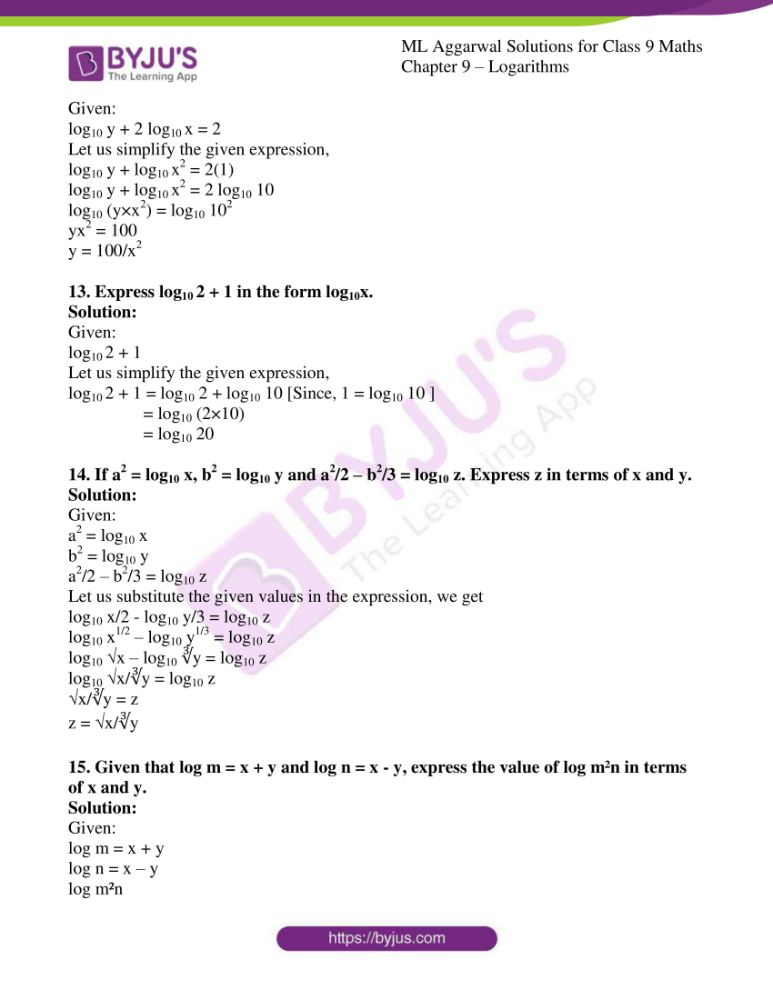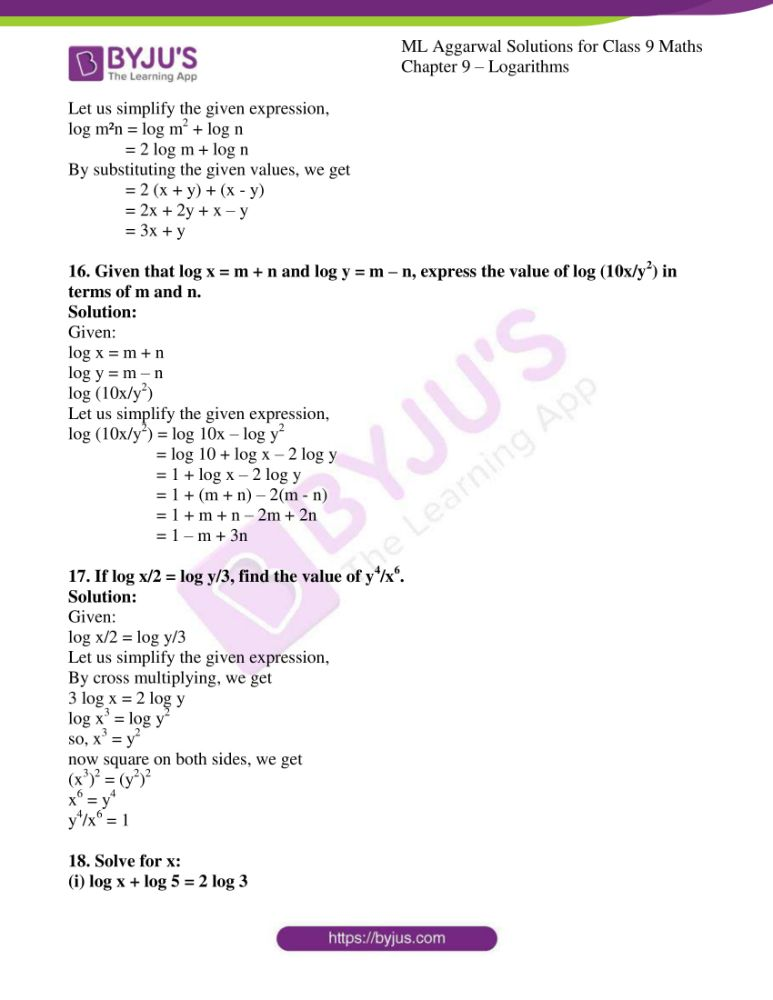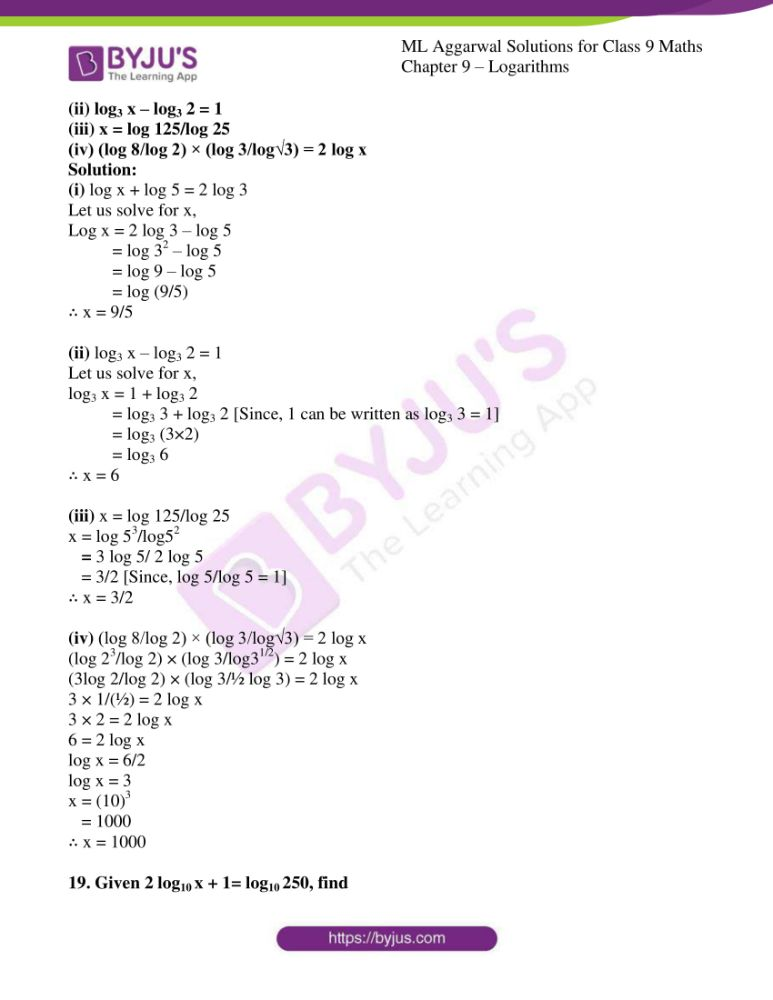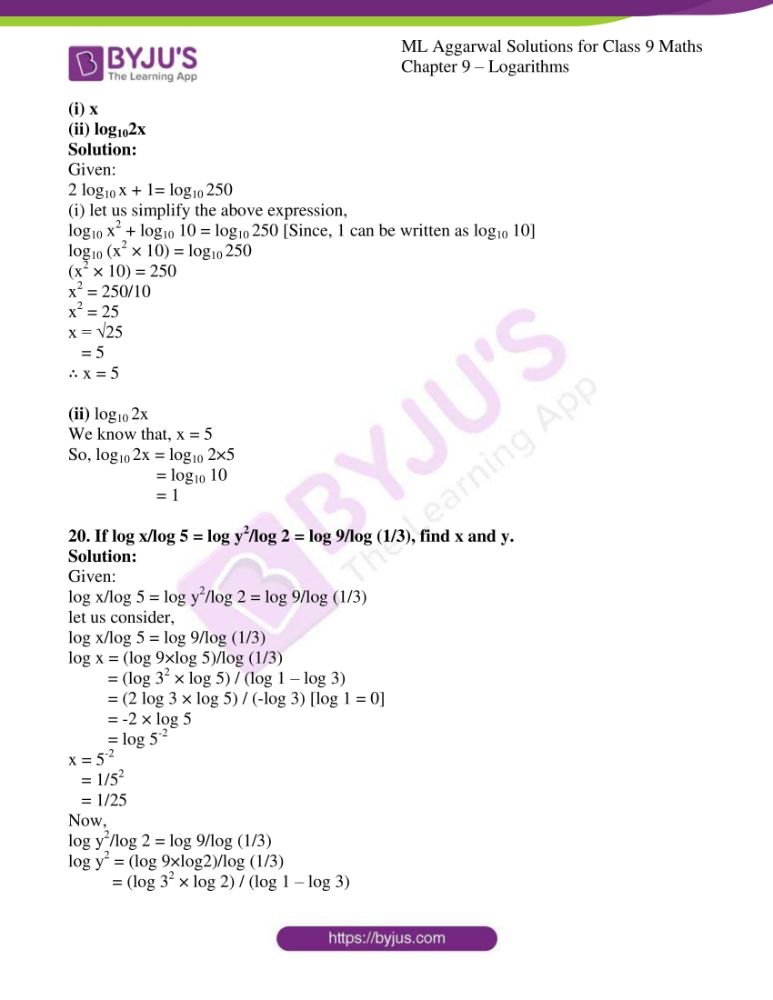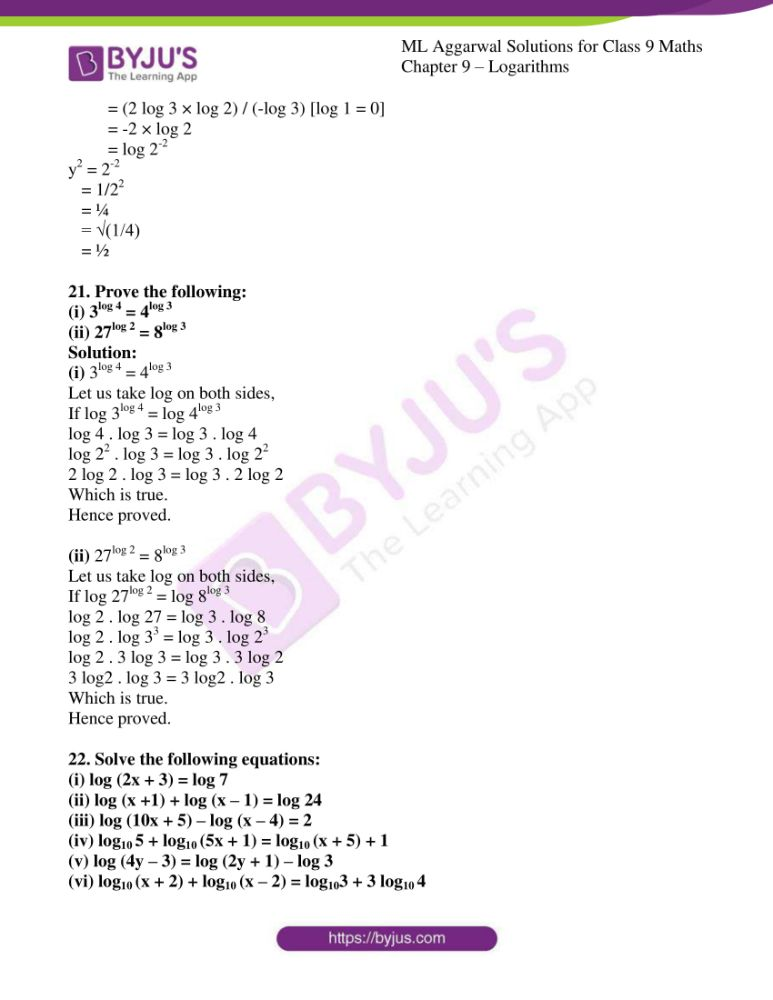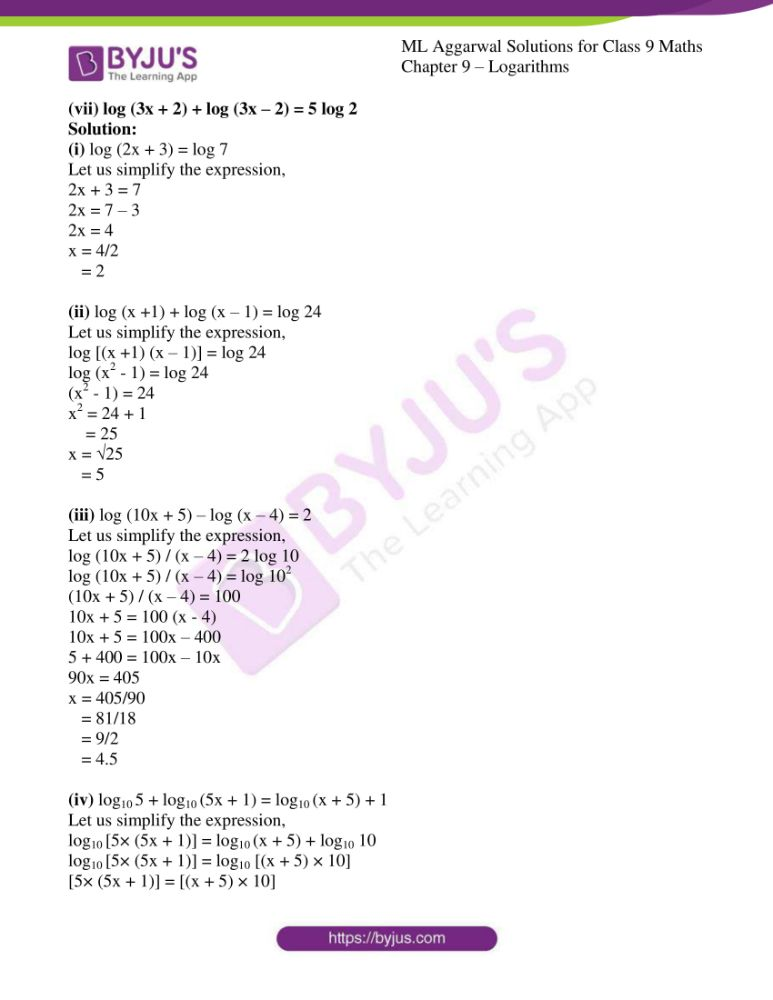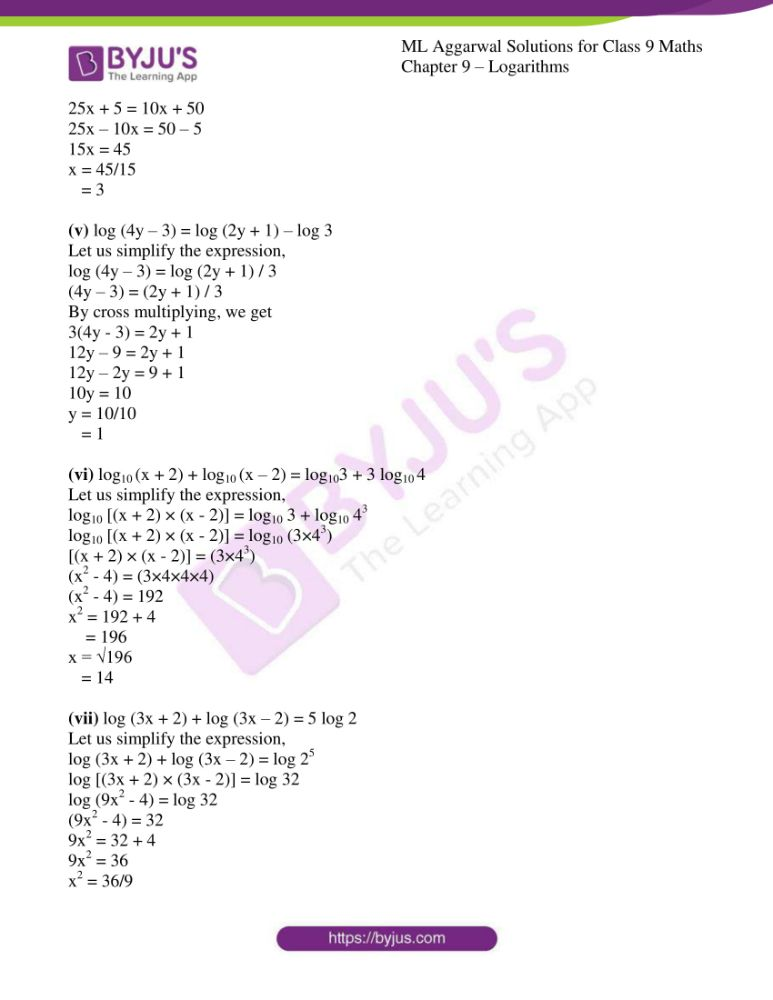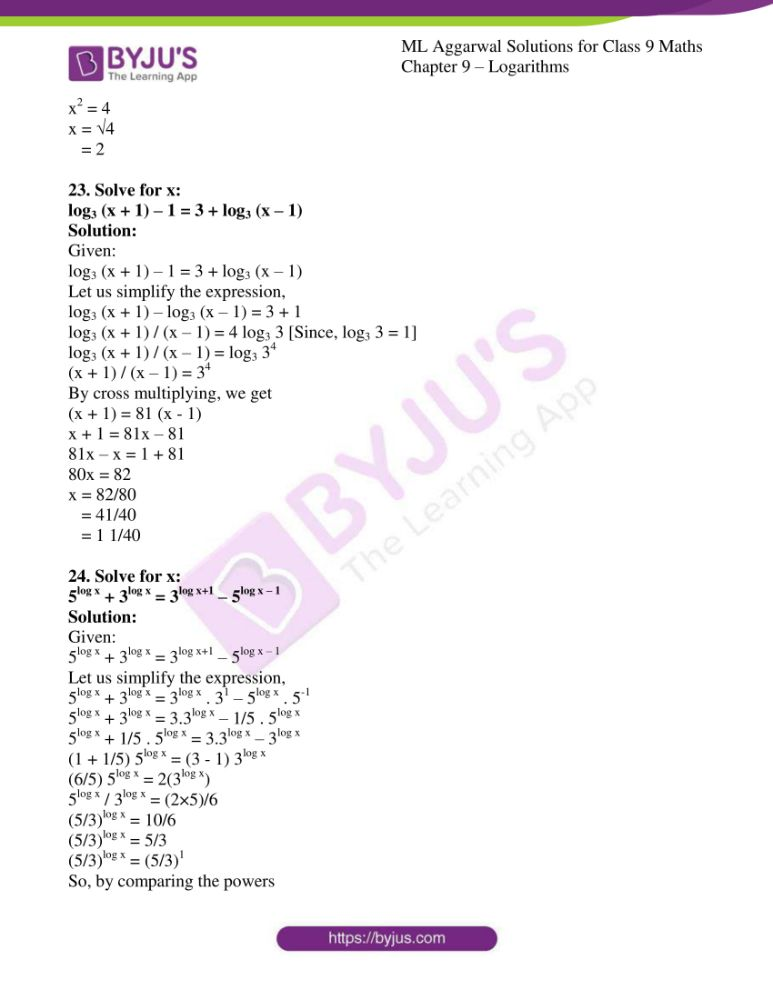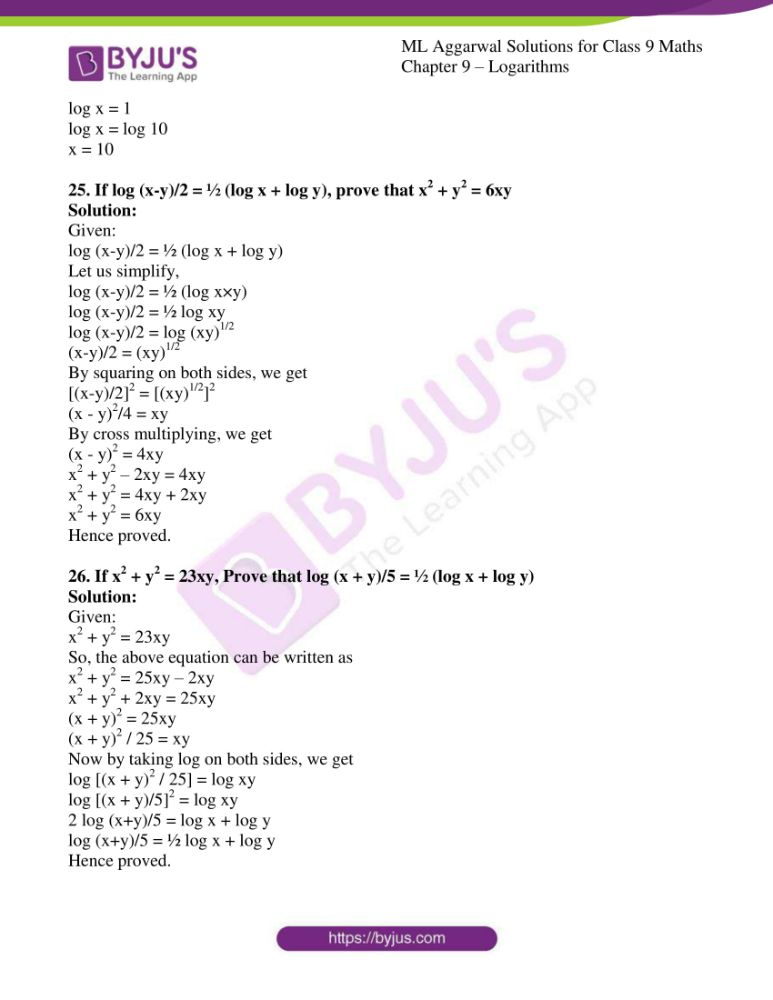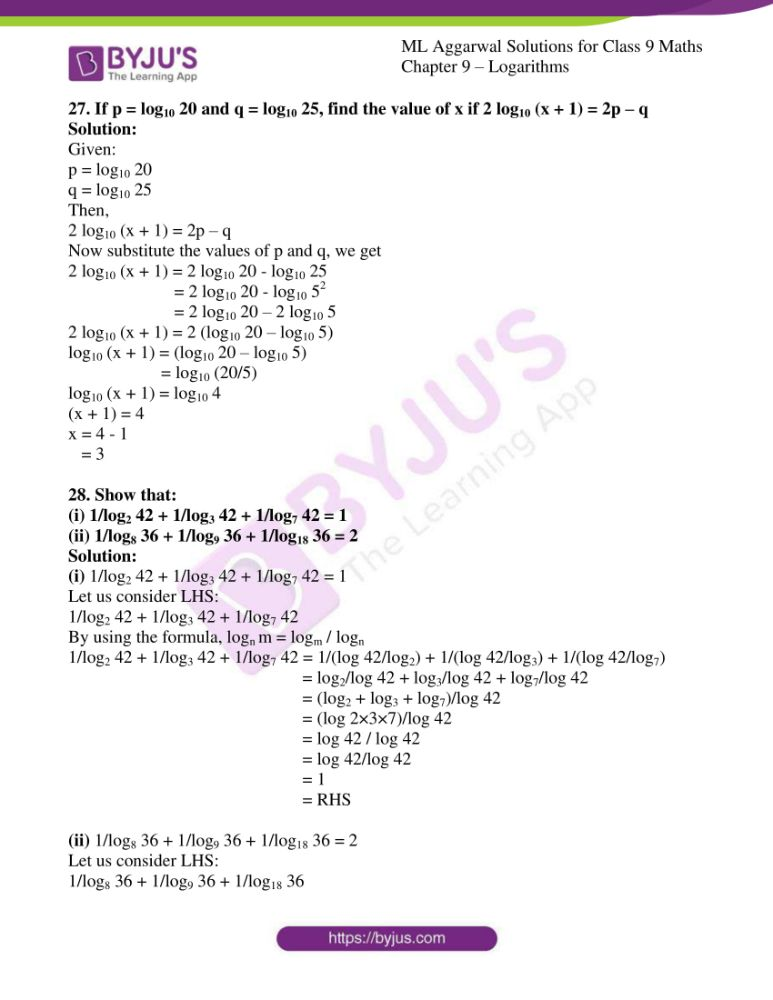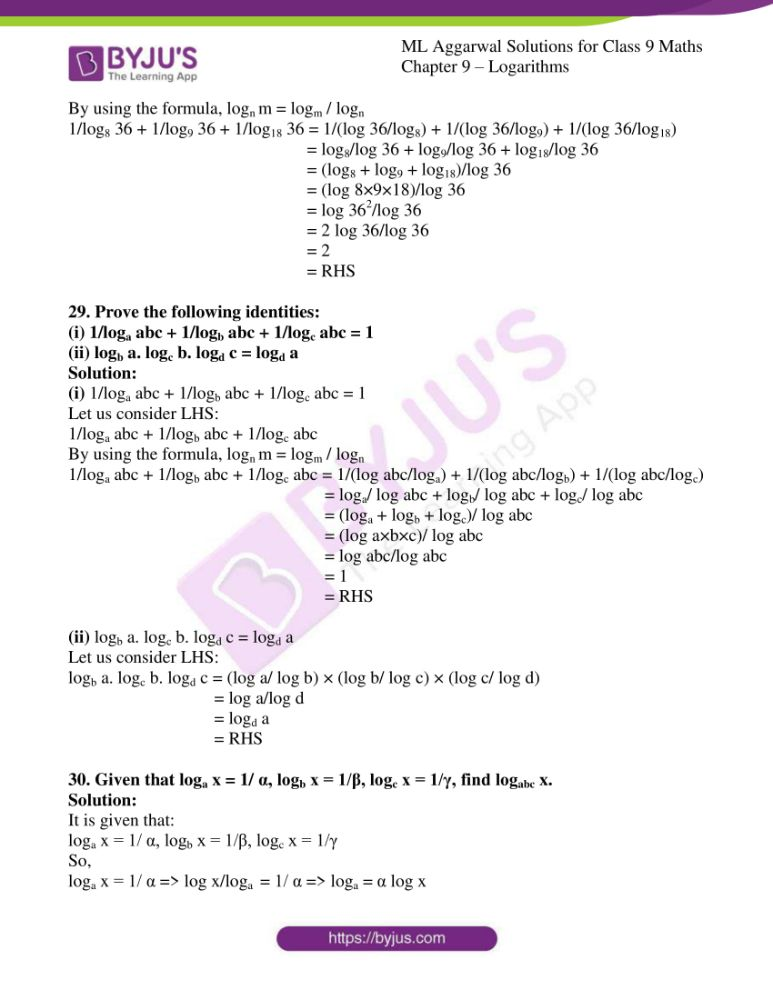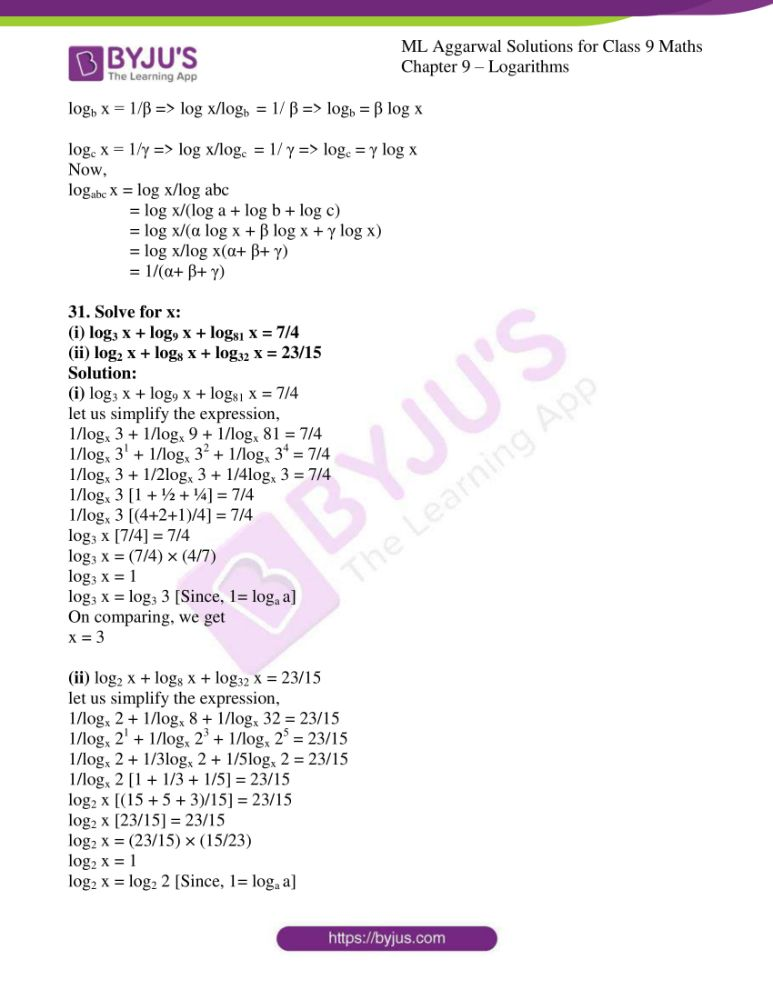### Access answers to ML Aggarwal Solutions for Class 9 Maths Chapter 9 – Logarithms

EXERCISE 9.1

1. Convert the following to logarithmic form:
(i) 52 = 25
(ii) a5 = 64
(iii) 7x = 100
(iv) 9o = 1
(v) 61 = 6
(vi) 3-2 = 1/9
(vii) 10-2 = 0.01
(viii) (81)3/4 = 27

Solution:

(i) 52 = 25

Let us apply log, we get

Log5 25 = 2

(ii) a5 = 64

Let us apply log, we get

Loga 64 = 5

(iii) 7x = 100

Let us apply log, we get

Log7 100 = x

(iv) 9o = 1

Let us apply log, we get

Log9 1 = 0

(v) 61 = 6

Let us apply log, we get

Log6 6 = 1

(vi) 3-2 = 1/9

Let us apply log, we get

Log3 1/9 = -2

(vii) 10-2 = 0.01

Let us apply log, we get

Log10 0.01 = -2

(viii) (81)3/4 = 27

Let us apply log, we get

Log81 27 = ¾

2. Convert the following into exponential form:

(i) log2 32 = 5

(ii) log3 81=4

(iii) log3 1/3 = -1

(iv) log3 4 = 2/3

(v) log8 32 = 5/3

(vi) log10 (0.001) = -3

(vii) log2 0.25 = -2

(viii) loga (1/a) = -1

Solution:

(i) log2 32 = 5

The exponential form of the expression is

25 = 32

(ii) log3 81=4

The exponential form of the expression is

34 = 81

(iii) log3 1/3 = -1

The exponential form of the expression is

3-1 = 1/3

(iv) log3 4 = 2/3

The exponential form of the expression is

(8)2/3 = 4

(v) log8 32 = 5/3

The exponential form of the expression is

(8)5/3 = 32

(vi) log10 (0.001) = -3

The exponential form of the expression is

10-3 = 0.001

(vii) log2 0.25 = -2

The exponential form of the expression is

2-2 = 0.25

(viii) loga (1/a) = -1

The exponential form of the expression is

a-1 = 1/a

3. By converting to exponential form, find the values of:

(i) log2 16

(ii) log5 125

(iii) log4 8

(iv) log9 27

(v) log10 (.01)

(vi) log7 1/7

(vii) log.5 256

(viii) log2 0.25

Solution:

(i) log2 16

Let us consider log2 16 = x

So,

(2)x = 16

= 2 × 2 × 2 × 2

2x = 24

By comparing the powers,

x = 4

(ii) log5 125

Let us consider log5 125 = x

So,

(5)x = 125

= 5 × 5 × 5

5x = 53

By comparing the powers,

x = 3

(iii) log4 8

Let us consider log4 8 = x

So,

(4)x = 8

(2 × 2)x = 2 × 2 × 2

22x = 23

By comparing the powers,

2x = 3

x = 3/2

(iv) log9 27

Let us consider log9 27= x

So,

(9)x = 27

(3 × 3)x = 3 × 3 × 3

32x = 33

By comparing the powers,

2x = 3

x = 3/2

(v) log10 (.01)

Let us consider log10 (.01) = x

So,

(10)x = 1/100

= 1/10 × 1/10

10x = 1/(10)2

10x = 10-2

By comparing the powers,

x = -2

(vi) log7 1/7

Let us consider log7 1/7 = x

So,

(7)x = 1/7

7x = 7-1

By comparing the powers,

x = -1

(vii) log.5 256

Let us consider log.5 256 = x

So,

(.5)x = 256

(5/10)x = 2 × 2 × 2 × 2 × 2 × 2 × 2 × 2

(1/2)x = 28

(2)-x = 28

By comparing the powers,

-x = 8

x = -8

(viii) log2 0.25

Let us consider log2 0.25 = x

So,

(2)x = 0.25

= 25/100

2x = 1/4

2x = (2)-2

By comparing the powers,

x = -2

4. Solve the following equations for x:

(i) log3 x = 2

(ii) logx 25 = 2

(iii) log10 x = -2

(iv) log4 x = ½

(v) logx 11 = 2.5

(vi) logx ¼ = -1

(vii) log81 x = 3/2

(viii) log9 x = 2.5

(ix) log4 x = -1.5

Solution:

(i) log3 x = 2

Let us simplify the expression,

(3)2 = x

x = 9

(ii) logx 25 = 2

Let us simplify the expression,

(x)2 = 25

= 5 × 5

x2 = 52

Since the powers are same,

So,

x = 5

(iii) log10 x = -2

Let us simplify the expression,

(10)-2 = x

x = 1/(10)2

= 1/100

x = 0.01

(iv) log4 x = ½

Let us simplify the expression,

(4)1/2 = x

x = (2 × 2)1/2

= (2)2×1/2

x = 2

(v) logx 11 = 2.5

Let us simplify the expression,

(x)1 = 11

x = 11

(vi) logx ¼ = -1

Let us simplify the expression,

(x)-1 = ¼

x-1 = 4-1

Since the powers are same,

So,

x = 4

(vii) log81 x = 3/2

Let us simplify the expression,

(81)3/2 = x

x = 813/2

= (34)3/2

= 34×3/2

= 36

= 3 × 3 × 3 × 3 × 3 × 3

= 729

x = 729

(viii) log9 x = 2.5

log9 x = 5/2

Let us simplify the expression,

(9)5/2 = x

x = (32)5/2

= 32×5/2

= 35

= 3 × 3 × 3 × 3 × 3

= 234

x = 234

(ix) log4 x = -1.5

log4 x = -3/2

Let us simplify the expression,

(4)-3/2 = x

x = (22)-3/2

= 22×-3/2

= 2-3

= 1/23

= 1/(2 × 2 × 2)

= 1/8

x = 1/8

5. Given log10 a = b, express 102b-3 in terms of a.

Solution:

Given:

log10 a = b

(10)b = a

Now,

102b-3 = (10)2b / (10)3

= (10b)2 / (10 × 10 × 10)

Substitute the value of (10)b = a, we get

= a2/1000

6. Given log10 x= a, log10 y = b and log10 z =c,

(i) write down 102a-3 in terms of x.

(ii) write down 103b-1 in terms of y.

(iii) if log10 P = 2a + b/2 – 3c, express P in terms of x, y and z.

Solution:

Given:

log10 x= a

• (10)a = x

log10 y = b

• (10)b = y

log10 z =c

• (10)c = z

(i) Write down 102a-3 in terms of x.

102a-3 = (10)2a / (10)3

= (10a)2 / (10 × 10 × 10)

Substitute the value of (10)a = x, we get

= x2/1000

(ii) Write down 103b-1 in terms of y.

103b-1 = (10)3b / (10)1

= (10b)3 / (10)

Substitute the value of (10)b = y, we get

= y3/10

(iii) If log10 P = 2a + b/2 – 3c, express P in terms of x, y and z.

we know that,

(10)a = x

(10)b = y

(10)c = z

By substituting the values

log10 P = 2a + b/2 – 3c

= 2 log10 x + ½ log10 y – 3 log10 z

= log10 x2 + log10 y1/2 – log10 z3

= log10 (x2 + y1/2) – log10 z3

= log10 [(x2y)/z3]

P = (x2y)/z3

7. If log10x = a and log10y = b, find the value of xy.

Solution:

Given:

log10x = a

(10)a = x

log10y = b

(10)b = y

Then,

xy = (10)a × (10)b

= (10)a+b

8. Given log10 a = m and log10 b = n, express a3/b2 in terms of m and n.

Solution:

Given:

log10 a = m

(10)m = a

log10 b = n

(10)n = b

So,

a3/b2 = (10m)3 / (10n)2

= 103m / 102n

= 103m – 2n

9. Given log10a= 2a and log10y = –b/2

(i) write 10a in terms of x.

(ii) write 102b+1 in terms of y.

(iii) if log10P= 3a – 2b, express P in terms of x and y.

Solution:

Given:

log10a= 2a

(10)2a = a

log10y = –b/2

(10)-b/2 = y

(i) Write 10a in terms of x.

10a = (102a)1/2

= (x)1/2

= x

(ii) Write 102b+1 in terms of y.

102b+1 = 102b × 101

= 104(b/2) × 101

= (10b/2)4 × 101

= y4 × 101

= 10y4

(iii) If log10P= 3a – 2b, express P in terms of x and y.

log10P= 3a – 2b

Substitute the values,

log10P= 3/2 (2a) – 4(b/2)

= 3/2 (log10 x) – 4 (log10 y)

= (log10 x)3/2 – (log10 y)4

= log10 [(x3/2) / y4]

P = (x3/2) / y4

10. If log2 y = x and log3 z = x, find 72x in terms of y and z.

Solution:

Given:

log2 y = x

2x = y

log3 z = x

3x = z

So,

72x = (2 × 2 × 2 × 3 × 3)x

= (23 × 32)x

= 23x × 32x

= (2x)3 × (3x)2

= y3 × z2

= y3z2

11. If log2 x = a and log5y = a, write 1002a-1 in terms of x and y.

Solution:

Given:

log2 x = a

2a = x

log5y = a

5a = y

So,

1002a-1 = (2 × 2 × 5 × 5)2a-1

= (22 × 52)2a-1

= 24a-2 × 5a-2

= (24a)/22 × (54a)/52

= [(2a)4 × (5a)4] / (4×25)

= (x4y4)/100

EXERCISE 9.2

1. Simplify the following:

(i) log a3 – log a2

(ii) log a3 ÷ log a2

(iii) log 4/log 2

(iv) (log 8 log 9)/log 27

(v) log 27/log √3

(vi) (log 9 – log 3)/log 27

Solution:

(i) log a3 – log a2

By using Quotient law,

log a3 – log a2 = log (a3/a2)

= log a

(ii) log a3 ÷ log a2

By using power law,

log a3 ÷ log a2 = 3log a ÷ 2 log a

= 3log a / 2log a

= 3/2

(iii) log 4/log 2

Let us simplify the expression,

log 4/log 2 = log(2×2)/log 2

By using power law,

= 2 log 2/log 2

= 2

(iv) (log 8 log 9)/log 27

Let us simplify the expression,

(log 8 log 9)/log 27 = (log 23. log 32)/log 33

By using power law,

= [(3 log 2).(2 log 3)]/(3 log 3)

= [(log 2).2] / 1

= 2 log 2

= log 22

= log 4

(v) log 27/log √3

Let us simplify the expression,

log 27/log √3 = log(3×3×3)/log(3)1/2

= log 33/log 31/2

By using power law

= 3log 3/((1/2)log 3)

= (3×2)/1 (log 3/log 3)

= (6) (1)

= 6

(vi) (log 9 – log 3)/log 27

Let us simplify the expression,

(log 9 – log 3)/log 27 = [log(3×3) – log 3] / log(3×3×3)

= [log 32 – log 3] / log 33

By using power law

= [2 log 3 – log 3]/3log 3

= log 3/3log 3

= 1/3

2. Evaluate the following:

(i) log (10 ÷ ∛10)

(ii) 2 + ½ log(10-3)

(iii) 2 log 5 + log 8 – ½ log 4

(iv) 2 log 103 + 3 log 10-2 – 1/3 log 5-3 + ½ log 4

(v) 2 log 2 + log 5 – ½ log 36 – log 1/30

(vi) 2 log 5 + log 3 + 3 log 2 – ½ log 36 – 2 log 10

(vii) log 2 + 16 log 16/15 + 12 log 25/24 + 7 log 81/80

(viii) 2 log10 5 + log10 8 – ½ log10 4

Solution:

(i) log (10 ÷ ∛10)

Let us simplify the expression,

log (10 ÷ ∛10) = log (10 ÷ 101/3)

= log (101 – 1/3)

= log (102/3)

= 2/3 log 10

= 2/3 (1)

= 2/3

(ii) 2 + ½ log(10-3)

Let us simplify the expression,

2 + ½ log(10-3) = 2 + ½ × (-3) log 10

= 2 – 3/2 log 10

= 2 – 3/2 (1)

= 2 – 3/2

= (4-3)/2

= ½

(iii) 2 log 5 + log 8 – ½ log 4

Let us simplify the expression,

2 log 5 + log 8 – ½ log 4 = log 52 + log 8 – ½ log 22

= log 25 + log 8 – ½ 2log 2

= log 25 + log 8 – log 2

= log (25×8)/2

= log (25×4)

= log 100

= log 102

= 2 log 10

= 2 (1)

= 2

(iv) 2 log 103 + 3 log 10-2 – 1/3 log 5-3 + ½ log 4

Let us simplify the expression,

2 log 103 + 3 log 10-2 – 1/3 log 5-3 + ½ log 4 = 2×3 log 10 + 3(-2)log 10 – 1/3 (-3) log 5 + ½ log 22

= 6 log 10 – 6 log 10 + log 5 + ½ 2 log 2

= 6 log 10 – 6 log 10 + log 5 + log 2

= 0 + log 5 + log 2

= log (5×2)

= log 10

= 1

(v) 2 log 2 + log 5 – ½ log 36 – log 1/30

Let us simplify the expression,

2 log 2 + log 5 – ½ log 36 – log 1/30 = log 22 + log 5 – ½ log 62 – log (1/30)

= log 4 + log 5 – log 6 – log 1/30

= log 4 + log 5 – log 6 – (log 1 – log 30)

= log 4 + log 5 – log 6 – log 1 + log 30

= log 4 + log 5 + log 30 – (log 6 + log 1)

= log (4×5×30) – log (6×1)

= log (4×5×30)/(6×1)

= log (4×5×5)

= log 100

= log 102

= 2 log 10

= 2 (1)

= 2

(vi) 2 log 5 + log 3 + 3 log 2 – ½ log 36 – 2 log 10

Let us simplify the expression,

2log 5 + log 3 + 3log 2 – ½ log 36 – 2log 10 = log 52 + log 3 + log 23 – ½ log 62 – log 102

= log 25 + log 3 + log 8 – log 6 – log 100

= log (25×3×8) – log(6×100)

= log (25×3×8)/ (6×100)

= log (1×3×8) / (6×4)

= log 24/24

= log 1

= 0

(vii) log 2 + 16 log 16/15 + 12 log 25/24 + 7 log 81/80

Let us simplify the expression,

log 2 + 16 log 16/15 + 12 log 25/24 + 7 log 81/80 = log 2 + 16(log 16 – log 15) + 12(log 25 – log 24) + 7(log 81 – log 80)

= log 2 + 16 (log 24 – log (3×5)) + 12 (log 52 – log (3×2×2×2)) + 7 (log (3×3×3×3) – log (24 × 5))

= log 2 + 16(4log 2 – (log 3 + log 5)) + 12 (2log 5 – log (3×23)) + 7 (log 34 – (log 24 + log 5))

= log 2 + 16 (4log 2 – log 3 – log 5) + 12 (2log 5 – (log 3 + 3log 2)) + 7 (4log 3 – 4log 2 – log 5)

= log 2 + 64log 2 – 16log 3 – 16log 5 + 24log 5 – 12log 3 – 36log 2 + 28log 3 – 28log 2 – 7log 5

= (log 2 + 64log 2 – 36log 2 – 28log 2) + (-16log 3 – 12log 3 + 28log 3) + (-16log 5 + 24log 5 – 7log 5)

= (65log 2 – 64log 2) + (-28log 3 + 28log 3) + (-23log 5 + 24log 5)

= log 2 + 0 + log 5

= log (2×5)

= log 10

= 1

(viii) 2 log10 5 + log10 8 – ½ log10 4

Let us simplify the expression,

2 log10 5 + log10 8 – ½ log10 4 = log10 52 + log10 8 – log10 41/2

= log10 25 + log10 8 – log10 (2)2×1/2

= log10 25 + log10 8 – log10 2

= log10 [(25×8)/2]

= log10 (25×4)

= log10 100

= log10 102

= 2 log10 10

= 2 (1)

= 2

3. Express each of the following as a single logarithm:

(i) 2 log 3 – ½ log 16 + log 12

(ii) 2 log10 5 – log10 2 + 3 log10 4 + 1

(iii) ½ log 36 + 2 log 8 – log 1.5

(iv) ½ log 25 – 2 log 3 + 1

(v) ½ log 9 + 2 log 3 – log 6 + log 2 – 2

Solution:

(i) 2 log 3 – ½ log 16 + log 12

Let us simplify the expression into single logarithm,

2 log 3 – ½ log 16 + log 12 = 2 log 3 – ½ log 42 + log 12

= 2 log 3 – log 4 + log 12

= log 32 – log 4 + log 12

= log 9 – log 4 + log 12

= log (9×12)/4

= log (9×3)

= log 27

(ii) 2 log10 5 – log10 2 + 3 log10 4 + 1

Let us simplify the expression into single logarithm,

2 log10 5 – log10 2 + 3 log10 4 + 1 = log10 52 – log10 2 + log10 43 + log10 10

= log10 25 – log10 2 + log10 64 + log10 10

= log10 (25×64×10) – log10 2

= log10 (16000) – log10 2

= log10 (16000/2)

= log10 8000

(iii) ½ log 36 + 2 log 8 – log 1.5

Let us simplify the expression into single logarithm,

½ log 36 + 2 log 8 – log 1.5 = log 361/2 + log 82 – log 1.5

= log (6)2×1/2 + log 64 – log 1.5

= log 6 + log 64 – log (15/10)

= log 6 + log 64 – (log 15 – log 10)

= log (6×64) – log 15 + log 10

= log (6×64×10) – log 15

= log [(6×64×10)/15]

= log (4×64)

= log 256

(iv) ½ log 25 – 2 log 3 + 1

Let us simplify the expression into single logarithm,

½ log 25 – 2 log 3 + 1 = log 251/2 – log 32 + log 10

= log (5)2×1/2 – log 9 + log 10

= log 5 – log 9 + log 10

= log (5×10) – log 9

= log ((5×10)/9)

= log 50/9

(v) ½ log 9 + 2 log 3 – log 6 + log 2 – 2

Let us simplify the expression into single logarithm,

½ log 9 + 2 log 3 – log 6 + log 2 – 2 = log 91/2 + log 32 – log 6 + log 2 – log 100

= log 32×1/2 + log 9 – log 6 + log 2 – log 100

= log 3 + log 9 – log 6 + log 2 – log 100

= log [(3×9×2)/(6×100)]

= log 9/100

4. Prove the following:

(i) log10 4 ÷ log10 2 = log3 9

(ii) log10 25 + log10 4 = log5 25

Solution:

(i) log10 4 ÷ log10 2 = log3 9

Let us consider LHS, log10 4 ÷ log10 2

log10 4 ÷ log10 2 = log10 22 ÷ log10 2

= 2 log10 2 ÷ log10 2

= 2 log10 2/ log10 2

= 2 (1)

= 2

Now let us consider RHS,

log3 9 = log3 32

= 2 log3 3

= 2(1)

= 2

∴ LHS = RHS

Hence proved.

(ii) log10 25 + log10 4 = log5 25

Let us consider LHS, log10 25 + log10 4

log10 25 + log10 4 = log10 (25×4)

= log10 100

= log10 102

= 2 log10 10

= 2(1)

= 2

Now, let us consider RHS,

log5 25 = log5 52

= 2 log5 5

= 2 (1)

= 2

∴ LHS = RHS

Hence proved.

5. If x = (100)a, y = (10000)b and z = (10)c, express log [(10√y)/x2z3] in terms of a, b, c.

Solution:

Given:

x = (100)a = (102)a = 102a

y = (10000)b = (104)b = 104b

z = (10)c

It is given that, log [(10√y)/x2z3]

log [(10√y)/x2z3] = (log 10 + log √y) – (log x2 + log z3)

= (1 + log(y)1/2) – (log x2 + log z3) [we know that, log 10 = 1]

= (1 + ½ log y) – (2 log x + 3 log z)

Now substitute the values of x, y, z, we get

= (1 + ½ log 104b) – (2 log 102a + 3 log 10c)

= (1 + ½ 4b log 10) – (2×2a log 10 + 3×c log 10)

= (1 + ½ 4b) – (2×2a + 3c) [Since, log 10 = 1]

= (1 + 2b) – (4a + 3c)

= 1 + 2b – 4a – 3c

6. If a = log10x, find the following in terms of a :

(i) x

(ii) log10 5√x2

(iii) log10 5x

Solution:

Given:

a = log10x

(i) x

10a = x

∴ x = 10a

(ii) log10 5√x2

log10 5√x2 = log10 (x2)1/5

= log10 (x)2/5

= 2/5 log10 x

= 2/5 (a)

= 2a/5

(iii) log10 5x

x = (10)a

= log10 5x

= log10 5(10)a

= log10 5 + log10 10

= log10 5 + a(1)

= a + log10 5

7. If a =log 2/3, b = log 3/5 and c = 2 log √(5/2). Find the value of

(i) a + b + c

(ii) 5a+b+c

Solution:

Given:

a =log 2/3

b = log 3/5

c = 2 log √(5/2)

(i) a + b + c

Let us substitute the given values, we get

a + b + c = log 2/3 + log 3/5 + 2 log √(5/2)

= (log 2 – log 3) + (log 3 – log 5) + 2 log (5/2)1/2

= log 2 – log 3 + log 3 – log 5 + 2 × ½ (log 5 – log 2)

= log 2 – log 3 + log 3 – log 5 + log 5 – log 2

= 0

(ii) 5a+b+c

5a+b+c = 50

= 1

8. If x = log 3/5, y = log 5/4 and z = 2 log √3/2, find the value of

(i) x + y – z

(ii) 3x+y-z

Solution:

Given:

x = log 3/5 = log 3 – log 5

y = log 5/4 = log 5 – log 4

z = 2 log √3/2 = log (√3/2)2 = log ¾ = log 3 – log 4

(i) x + y – z

Let us substitute the given values, we get

x + y – z = log 3 – log 5 + log 5 – log 4 – (log 3 – log 4)

= log 3 – log 5 + log 5 – log 4 – log 3 + log 4

= 0

(ii) 3x+y-z

3x+y-z = 30

= 1

9. If x = log10 12, y = log4 2 × log10 9 and z = log10 0.4, find the values of

(i) x – y – z

(ii) 7x-y-z

Solution:

Given:

x = log10 12

y = log4 2 × log10 9

z = log10 0.4

(i) x – y – z

Let us substitute the given values, we get

x – y – z = log10 12 – log4 2 × log10 9 – log10 0.4

= log10 (3×4) – log4 41/2 × log10 32 – log10 4/10

= log10 3 + log10 4 – ½ log4 4 × 2 log10 3 – (log10 4 – log10 10)

= log10 3 + log10 4 – ½ × 1 × 2 log10 3 – log10 4 + 1

= log10 3 + log10 4 – log10 3 – log10 4 + 1

= 1

(ii) 7x-y-z

7x-y-z = 71

= 7

10. If log V + log 3 = log π + log 4 + 3 log r, find V in terms of other quantities.
Solution:

Given:

log V + log 3 = log π + log 4 + 3 log r

Let us simplify the given expression to find V,

log (V × 3) = log (π × 4 × r3)

log 3V = log 4πr3

3V = 4πr3

V = 4πr3/3

11. Given 3 (log 5 – log 3) – (log 5 – 2 log 6) = 2 – log n, find n.

Solution:

Given:

3 (log 5 – log 3) – (log 5 – 2 log 6) = 2 – log n

Let us simplify the given expression to find n,

3 log 5 – 3 log 3 – log 5 + 2 log 6 = 2 – log n

2 log 5 – 3 log 3 + 2 log 6 = 2 (1) – log n

log 52 – log 33 + log 62 = 2 log 10 – log n [Since, 1 = log 10]

log 25 – log 27 + log 36 – log 102 = – log n

log n = – log 25 + log 27 – log 36 + log 100

= (log 100 + log 27) – (log 25 + log 36)

= log (100×27) – log (25×36)

= log (100×27)/(25×36)

log n = log 3

n = 3

12. Given that log10 y + 2 log10 x = 2, express y in terms of x.
Solution:

Given:

log10 y + 2 log10 x = 2

Let us simplify the given expression,

log10 y + log10 x2 = 2(1)

log10 y + log10 x2 = 2 log10 10

log10 (y×x2) = log10 102

yx2 = 100

y = 100/x2

13. Express log10 2 + 1 in the form log10x.

Solution:

Given:

log10 2 + 1

Let us simplify the given expression,

log10 2 + 1 = log10 2 + log10 10 [Since, 1 = log10 10 ]

= log10 (2×10)

= log10 20

14. If a2 = log10 x, b2 = log10 y and a2/2 – b2/3 = log10 z. Express z in terms of x and y.

Solution:

Given:

a2 = log10 x

b2 = log10 y

a2/2 – b2/3 = log10 z

Let us substitute the given values in the expression, we get

log10 x/2 – log10 y/3 = log10 z

log10 x1/2 – log10 y1/3 = log10 z

log10 x – log10 ∛y = log10 z

log10 x/∛y = log10 z

x/∛y = z

z = x/∛y

15. Given that log m = x + y and log n = x – y, express the value of log m²n in terms of x and y.

Solution:

Given:

log m = x + y

log n = x – y

log m²n

Let us simplify the given expression,

log m²n = log m2 + log n

= 2 log m + log n

By substituting the given values, we get

= 2 (x + y) + (x – y)

= 2x + 2y + x – y

= 3x + y

16. Given that log x = m + n and log y = m – n, express the value of log (10x/y2) in terms of m and n.

Solution:

Given:

log x = m + n

log y = m – n

log (10x/y2)

Let us simplify the given expression,

log (10x/y2) = log 10x – log y2

= log 10 + log x – 2 log y

= 1 + log x – 2 log y

= 1 + (m + n) – 2(m – n)

= 1 + m + n – 2m + 2n

= 1 – m + 3n

17. If log x/2 = log y/3, find the value of y4/x6.

Solution:

Given:

log x/2 = log y/3

Let us simplify the given expression,

By cross multiplying, we get

3 log x = 2 log y

log x3 = log y2

so, x3 = y2

now square on both sides, we get

(x3)2 = (y2)2

x6 = y4

y4/x6 = 1

18. Solve for x:

(i) log x + log 5 = 2 log 3

(ii) log3 x – log3 2 = 1

(iii) x = log 125/log 25

(iv) (log 8/log 2) × (log 3/log√3) = 2 log x

Solution:

(i) log x + log 5 = 2 log 3

Let us solve for x,

Log x = 2 log 3 – log 5

= log 32 – log 5

= log 9 – log 5

= log (9/5)

∴ x = 9/5

(ii) log3 x – log3 2 = 1

Let us solve for x,

log3 x = 1 + log3 2

= log3 3 + log3 2 [Since, 1 can be written as log3 3 = 1]

= log3 (3×2)

= log3 6

∴ x = 6

(iii) x = log 125/log 25

x = log 53/log52

= 3 log 5/ 2 log 5

= 3/2 [Since, log 5/log 5 = 1]

∴ x = 3/2

(iv) (log 8/log 2) × (log 3/log√3) = 2 log x

(log 23/log 2) × (log 3/log31/2) = 2 log x

(3log 2/log 2) × (log 3/½ log 3) = 2 log x

3 × 1/(½) = 2 log x

3 × 2 = 2 log x

6 = 2 log x

log x = 6/2

log x = 3

x = (10)3

= 1000

∴ x = 1000

19. Given 2 log10 x + 1= log10 250, find

(i) x

(ii) log102x
Solution:

Given:

2 log10 x + 1= log10 250

(i) let us simplify the above expression,

log10 x2 + log10 10 = log10 250 [Since, 1 can be written as log10 10]

log10 (x2 × 10) = log10 250

(x2 × 10) = 250

x2 = 250/10

x2 = 25

x = √25

= 5

∴ x = 5

(ii) log10 2x

We know that, x = 5

So, log10 2x = log10 2×5

= log10 10

= 1

20. If log x/log 5 = log y2/log 2 = log 9/log (1/3), find x and y.

Solution:

Given:

log x/log 5 = log y2/log 2 = log 9/log (1/3)

let us consider,

log x/log 5 = log 9/log (1/3)

log x = (log 9×log 5)/log (1/3)

= (log 32 × log 5) / (log 1 – log 3)

= (2 log 3 × log 5) / (-log 3) [log 1 = 0]

= -2 × log 5

= log 5-2

x = 5-2

= 1/52

= 1/25

Now,

log y2/log 2 = log 9/log (1/3)

log y2 = (log 9×log2)/log (1/3)

= (log 32 × log 2) / (log 1 – log 3)

= (2 log 3 × log 2) / (-log 3) [log 1 = 0]

= -2 × log 2

= log 2-2

y2 = 2-2

= 1/22

= ¼

= √(1/4)

= ½

21. Prove the following:
(i) 3log 4 = 4log 3
(ii) 27log 2 = 8log 3
Solution:

(i) 3log 4 = 4log 3

Let us take log on both sides,

If log 3log 4 = log 4log 3

log 4 . log 3 = log 3 . log 4

log 22 . log 3 = log 3 . log 22

2 log 2 . log 3 = log 3 . 2 log 2

Which is true.

Hence proved.

(ii) 27log 2 = 8log 3

Let us take log on both sides,

If log 27log 2 = log 8log 3

log 2 . log 27 = log 3 . log 8

log 2 . log 33 = log 3 . log 23

log 2 . 3 log 3 = log 3 . 3 log 2

3 log2 . log 3 = 3 log2 . log 3

Which is true.

Hence proved.

22. Solve the following equations:

(i) log (2x + 3) = log 7

(ii) log (x +1) + log (x – 1) = log 24

(iii) log (10x + 5) – log (x – 4) = 2

(iv) log10 5 + log10 (5x + 1) = log10 (x + 5) + 1

(v) log (4y – 3) = log (2y + 1) – log 3

(vi) log10 (x + 2) + log10 (x – 2) = log103 + 3 log10 4

(vii) log (3x + 2) + log (3x – 2) = 5 log 2

Solution:

(i) log (2x + 3) = log 7

Let us simplify the expression,

2x + 3 = 7

2x = 7 – 3

2x = 4

x = 4/2

= 2

(ii) log (x +1) + log (x – 1) = log 24

Let us simplify the expression,

log [(x +1) (x – 1)] = log 24

log (x2 – 1) = log 24

(x2 – 1) = 24

x2 = 24 + 1

= 25

x = √25

= 5

(iii) log (10x + 5) – log (x – 4) = 2

Let us simplify the expression,

log (10x + 5) / (x – 4) = 2 log 10

log (10x + 5) / (x – 4) = log 102

(10x + 5) / (x – 4) = 100

10x + 5 = 100 (x – 4)

10x + 5 = 100x – 400

5 + 400 = 100x – 10x

90x = 405

x = 405/90

= 81/18

= 9/2

= 4.5

(iv) log10 5 + log10 (5x + 1) = log10 (x + 5) + 1

Let us simplify the expression,

log10 [5× (5x + 1)] = log10 (x + 5) + log10 10

log10 [5× (5x + 1)] = log10 [(x + 5) × 10] [5× (5x + 1)] = [(x + 5) × 10]

25x + 5 = 10x + 50

25x – 10x = 50 – 5

15x = 45

x = 45/15

= 3

(v) log (4y – 3) = log (2y + 1) – log 3

Let us simplify the expression,

log (4y – 3) = log (2y + 1) / 3

(4y – 3) = (2y + 1) / 3

By cross multiplying, we get

3(4y – 3) = 2y + 1

12y – 9 = 2y + 1

12y – 2y = 9 + 1

10y = 10

y = 10/10

= 1

(vi) log10 (x + 2) + log10 (x – 2) = log103 + 3 log10 4

Let us simplify the expression,

log10 [(x + 2) × (x – 2)] = log10 3 + log10 43

log10 [(x + 2) × (x – 2)] = log10 (3×43)

[(x + 2) × (x – 2)] = (3×43)

(x2 – 4) = (3×4×4×4)

(x2 – 4) = 192

x2 = 192 + 4

= 196

x = √196

= 14

(vii) log (3x + 2) + log (3x – 2) = 5 log 2

Let us simplify the expression,

log (3x + 2) + log (3x – 2) = log 25

log [(3x + 2) × (3x – 2)] = log 32

log (9x2 – 4) = log 32

(9x2 – 4) = 32

9x2 = 32 + 4

9x2 = 36

x2 = 36/9

x2 = 4

x = √4

= 2

23. Solve for x:
log3 (x + 1) – 1 = 3 + log3 (x – 1)
Solution:

Given:

log3 (x + 1) – 1 = 3 + log3 (x – 1)

Let us simplify the expression,

log3 (x + 1) – log3 (x – 1) = 3 + 1

log3 (x + 1) / (x – 1) = 4 log3 3 [Since, log3 3 = 1]

log3 (x + 1) / (x – 1) = log3 34

(x + 1) / (x – 1) = 34

By cross multiplying, we get

(x + 1) = 81 (x – 1)

x + 1 = 81x – 81

81x – x = 1 + 81

80x = 82

x = 82/80

= 41/40

= 1 1/40

24. Solve for x:

5log x + 3log x = 3log x+1 – 5log x – 1

Solution:

Given:

5log x + 3log x = 3log x+1 – 5log x – 1

Let us simplify the expression,

5log x + 3log x = 3log x . 31 – 5log x . 5-1

5log x + 3log x = 3.3log x – 1/5 . 5log x

5log x + 1/5 . 5log x = 3.3log x – 3log x

(1 + 1/5) 5log x = (3 – 1) 3log x

(6/5) 5log x = 2(3log x)

5log x / 3log x = (2×5)/6

(5/3)log x = 10/6

(5/3)log x = 5/3

(5/3)log x = (5/3)1

So, by comparing the powers

log x = 1

log x = log 10

x = 10

25. If log (x-y)/2 = ½ (log x + log y), prove that x2 + y2 = 6xy

Solution:

Given:

log (x-y)/2 = ½ (log x + log y)

Let us simplify,

log (x-y)/2 = ½ (log x×y)

log (x-y)/2 = ½ log xy

log (x-y)/2 = log (xy)1/2

(x-y)/2 = (xy)1/2

By squaring on both sides, we get

[(x-y)/2]2 = [(xy)1/2]2

(x – y)2/4 = xy

By cross multiplying, we get

(x – y)2 = 4xy

x2 + y2 – 2xy = 4xy

x2 + y2 = 4xy + 2xy

x2 + y2 = 6xy

Hence proved.

26. If x2 + y2 = 23xy, Prove that log (x + y)/5 = ½ (log x + log y)

Solution:

Given:

x2 + y2 = 23xy

So, the above equation can be written as

x2 + y2 = 25xy – 2xy

x2 + y2 + 2xy = 25xy

(x + y)2 = 25xy

(x + y)2 / 25 = xy

Now by taking log on both sides, we get

log [(x + y)2 / 25] = log xy

log [(x + y)/5]2 = log xy

2 log (x+y)/5 = log x + log y

log (x+y)/5 = ½ log x + log y

Hence proved.

27. If p = log10 20 and q = log10 25, find the value of x if 2 log10 (x + 1) = 2p – q

Solution:

Given:

p = log10 20

q = log10 25

Then,

2 log10 (x + 1) = 2p – q

Now substitute the values of p and q, we get

2 log10 (x + 1) = 2 log10 20 – log10 25

= 2 log10 20 – log10 52

= 2 log10 20 – 2 log10 5

2 log10 (x + 1) = 2 (log10 20 – log10 5)

log10 (x + 1) = (log10 20 – log10 5)

= log10 (20/5)

log10 (x + 1) = log10 4

(x + 1) = 4

x = 4 – 1

= 3

28. Show that:

(i) 1/log2 42 + 1/log3 42 + 1/log7 42 = 1

(ii) 1/log8 36 + 1/log9 36 + 1/log18 36 = 2

Solution:

(i) 1/log2 42 + 1/log3 42 + 1/log7 42 = 1

Let us consider LHS:

1/log2 42 + 1/log3 42 + 1/log7 42

By using the formula, logn m = logm / logn

1/log2 42 + 1/log3 42 + 1/log7 42 = 1/(log 42/log2) + 1/(log 42/log3) + 1/(log 42/log7)

= log2/log 42 + log3/log 42 + log7/log 42

= (log2 + log3 + log7)/log 42

= (log 2×3×7)/log 42

= log 42 / log 42

= log 42/log 42

= 1

= RHS

(ii) 1/log8 36 + 1/log9 36 + 1/log18 36 = 2

Let us consider LHS:

1/log8 36 + 1/log9 36 + 1/log18 36

By using the formula, logn m = logm / logn

1/log8 36 + 1/log9 36 + 1/log18 36 = 1/(log 36/log8) + 1/(log 36/log9) + 1/(log 36/log18)

= log8/log 36 + log9/log 36 + log18/log 36

= (log8 + log9 + log18)/log 36

= (log 8×9×18)/log 36

= log 362/log 36

= 2 log 36/log 36

= 2

= RHS

29. Prove the following identities:

(i) 1/loga abc + 1/logb abc + 1/logc abc = 1

(ii) logb a. logc b. logd c = logd a

Solution:

(i) 1/loga abc + 1/logb abc + 1/logc abc = 1

Let us consider LHS:

1/loga abc + 1/logb abc + 1/logc abc

By using the formula, logn m = logm / logn

1/loga abc + 1/logb abc + 1/logc abc = 1/(log abc/loga) + 1/(log abc/logb) + 1/(log abc/logc)

= loga/ log abc + logb/ log abc + logc/ log abc

= (loga + logb + logc)/ log abc

= (log a×b×c)/ log abc

= log abc/log abc

= 1

= RHS

(ii) logb a. logc b. logd c = logd a

Let us consider LHS:

logb a. logc b. logd c = (log a/ log b) × (log b/ log c) × (log c/ log d)

= log a/log d

= logd a

= RHS

30. Given that loga x = 1/ α, logb x = 1/β, logc x = 1/γ, find logabc x.

Solution:

It is given that:

loga x = 1/ α, logb x = 1/β, logc x = 1/γ

So,

loga x = 1/ α => log x/loga = 1/ α => loga = α log x

logb x = 1/β => log x/logb = 1/ β => logb = β log x

logc x = 1/γ => log x/logc = 1/ γ => logc = γ log x

Now,

logabc x = log x/log abc

= log x/(log a + log b + log c)

= log x/(α log x + β log x + γ log x)

= log x/log x(α+ β+ γ)

= 1/(α+ β+ γ)

31. Solve for x:

(i) log3 x + log9 x + log81 x = 7/4

(ii) log2 x + log8 x + log32 x = 23/15

Solution:

(i) log3 x + log9 x + log81 x = 7/4

let us simplify the expression,

1/logx 3 + 1/logx 9 + 1/logx 81 = 7/4

1/logx 31 + 1/logx 32 + 1/logx 34 = 7/4

1/logx 3 + 1/2logx 3 + 1/4logx 3 = 7/4

1/logx 3 [1 + ½ + ¼] = 7/4

1/logx 3 [(4+2+1)/4] = 7/4

log3 x [7/4] = 7/4

log3 x = (7/4) × (4/7)

log3 x = 1

log3 x = log3 3 [Since, 1= loga a]

On comparing, we get

x = 3

(ii) log2 x + log8 x + log32 x = 23/15

let us simplify the expression,

1/logx 2 + 1/logx 8 + 1/logx 32 = 23/15

1/logx 21 + 1/logx 23 + 1/logx 25 = 23/15

1/logx 2 + 1/3logx 2 + 1/5logx 2 = 23/15

1/logx 2 [1 + 1/3 + 1/5] = 23/15

log2 x [(15 + 5 + 3)/15] = 23/15

log2 x [23/15] = 23/15

log2 x = (23/15) × (15/23)

log2 x = 1

log2 x = log2 2 [Since, 1= loga a]

On comparing, we get

x = 2